• 机器学习算法实例
千次阅读
2020-12-17 14:47:57

本文整理了60个机器学习算法应用场景实例，含分类算法应用场景20个、回归算法应用场景20个、聚类算法应用场景10个以及关联规则应用场景10个。包含了天池、DataCastle、DataFountain中所有竞赛场景。

## 1 分类算法应用场景实例

1.1 O2O优惠券使用预测

1.2 市民出行选乘公交预测

1.3待测微生物种类判别

1.4 基于运营商数据的个人征信评估

1.5 商品图片分类

1.6 广告点击行为预测

1.7 基于文本内容的垃圾短信识别

1.8 中文句子类别精准分析

1.9 P2P网络借贷平台的经营风险量化分析

1.10 国家电网客户用电异常行为分析

1.11 自动驾驶场景中的交通标志检测

1.12 大数据精准营销中搜狗用户画像挖掘

1.13 基于视角的领域情感分析

1.14 监控场景下的行人精细化识别

1.15 用户评分预测

1.16 猫狗识别大战

1.17 微额借款用户人品预测

1.18 验证码识别

1.19 客户流失率预测

1.20 汽车4S店邮件营销方案

## 2回归算法应用场景实例

2.1 机场客流量分布预测

2.2 音乐流行趋势预测

2.3 需求预测与仓储规划方案

2.4 新浪微博互动量预测

2.5 货币基金资金流入流出预测

2.6 电影票房预测

2.7 农产品价格预测分析

2.8 基于多源数据的青藏高原湖泊面积预测

2.9 微博传播规模和传播深度预测

2.10 鲍鱼年龄预测

2.11 学生成绩排名预测

2.12 网约车出行流量预测

2.13 红酒品质评分

2.14搜索引擎的搜索量和股价波动

2.15 中国人口增长分析

2.16 农村居民收入增长预测

2.17 房地产销售影响因素分析

2.18 股价走势预测

2.19 全国综合运输总量预测

2.20 地震预报

## 3聚类算法应用场景实例

3.1 基于用户位置信息的商业选址

3.2 中文地址标准化处理

3.3 国家电网用户画像

3.4 非人恶意流量识别

3.5 求职信息完善

3.6 搜索引擎查询聚类以进行流量推荐

3.7 生物种群固有结构认知

3.8 保险投保者分组

3.9 网站关键词来源聚类整和

3.10 图像分割

## 4关联规则应用场景实例

4.1 穿衣搭配推荐

4.2 互联网情绪指标和生猪价格的关联关系挖掘和预测

4.3 依据用户轨迹的商户精准营销

4.4 地点推荐系统

4.5 气象关联分析

4.6 交通事故成因分析

4.7 基于兴趣的实时新闻推荐

4.8 银行金融客户交叉销售分析

4.9 电子商务搭配购买推荐

4.10 银行营销方案推荐

## 1 分类算法应用场景实例

1.1 O2O优惠券使用预测

以优惠券盘活老用户或吸引新客户进店消费是O2O的一种重要营销方式。然而随机投放的优惠券对多数用户造成无意义的干扰。对商家而言，滥发的优惠券可能降低品牌声誉，同时难以估算营销成本。个性化投放是提高优惠券核销率的重要技术，它可以让具有一定偏好的消费者得到真正的实惠，同时赋予商家更强的营销能力。

现有O2O场景相关的丰富数据，希望通过分析建模，精准预测用户是否会在规定时间内使用相应优惠券。

1.2 市民出行选乘公交预测

基于海量公交数据记录，希望挖掘市民在公共交通中的行为模式。以市民出行公交线路选乘预测为方向，期望通过分析广东省部分公交线路的历史公交卡交易数据，挖掘固定人群在公共交通中的行为模式，分析推测乘客的出行习惯和偏好，从而建立模型预测人们在未来一周内将会搭乘哪些公交线路，为广大乘客提供信息对称、安全舒适的出行环境，用数据引领未来城市智慧出行。

1.3待测微生物种类判别

DNA是多数生物的遗传物质，DNA上的碱基（A，T，C和G）就储藏了遗传信息，不同物种的DNA序列在序列长度和碱基组成上差异显著。所以我们能够通过DNA序列的比较分析，来判断DNA序列是来自哪些物种。由于测序技术限制，我们只能得到一定长度的DNA序列片段。通过DNA序列片段与已知的微生物DNA序列进行比较，可以确定DNA片段的来源微生物，进而确定待测微生物种类。

期望在相关数据基础上，建立分析方法，在计算资源消耗尽量小的情况下，尽可能快地给出准确的结果，以满足临床诊断需求。

1.4 基于运营商数据的个人征信评估

运营商作为网络服务供应商，积累了大量的用户基本信息及行为特征数据，如终端数据、套餐消费数据、通信数据等等。实名制政策保证了运营商用户数据能与用户真实身份匹配，并真实客观的反映用户行为。广泛覆盖的网络基础设施提供了积累大量实时数据的条件，这些用户数据实时反馈着用户的各个维度的信息及特征。

在我国，个人征信评估主要通过引用央行个人征信报告，但对于很多用户没有建立个人信用记录的用户，金融机构想要了解他们的信用记录成本又较高，传统征信评估手段难以满足目前多种多样的新兴需求。金融业务不同于其他大数据业务，对数据的真实性、可信度和时效性要求较高，而这正是运营商数据的价值所在。

期望利用运营商用户数据，提供完善的个人征信评估。

1.5 商品图片分类

京东含有数以百万计的商品图片，“拍照购”“找同款”等应用必须对用户提供的商品图片进行分类。同时，提取商品图像特征，可以提供给推荐、广告等系统，提高推荐/广告的效果。

希望通过对图像数据进行学习，以达到对图像进行分类划分的目的。

1.6 广告点击行为预测

用户在上网浏览过程中，可能产生广告曝光或点击行为。对广告点击进行预测，可以指导广告主进行定向广告投放和优化，使广告投入产生最大回报。

希望基于100万名随机用户在六个月的时间范围内广告曝光和点击日志，包括广告监测点数据，预测每个用户在8天内是否会在各监测点上发生点击行为。

1.7 基于文本内容的垃圾短信识别

垃圾短信已日益成为困扰运营商和手机用户的难题，严重影响到人们正常生活、侵害到运营商的社会形象以及危害着社会稳定。而不法分子运用科技手段不断更新垃圾短信形式且传播途径非常广泛，传统的基于策略、关键词等过滤的效果有限，很多垃圾短信“逃脱”过滤，继续到达手机终端。

希望基于短信文本内容，结合机器学习算法、大数据分析挖掘来智能地识别垃圾短信及其变种。

1.8 中文句子类别精准分析

精确的语义分析是大数据必备技术，在分析句子时，不同句子类别即使用类似的关键词，表达的含义仍有很大差别，特别是在情感判断中。

希望通过新闻以及微博等文本数据，对其句子类别进行判断。

1.9 P2P网络借贷平台的经营风险量化分析

P2P网络借贷即点对点信贷，其风险情况始终触碰着投资人的神经。据网贷之家统计，截止今年9月份，出现问题的网贷平台一共有1008家，而仅仅今年就有641家平台出现问题，这说明了随着我国p2p行业的迅猛发展，P2P平台的风险预测已经成为一个至关重要的问题。P2P平台的风险主要是在运营过程中产生的，与运营数据有着密不可分的关系。P2P平台的风险预测并非无线索可寻，像借款期限和年化收益率等指标，就对P2P平台的风险预测有很重要的参考意义。

希望通过互联网数据，构建出P2P网贷平台的经营风险模型，从而能够比较准确的预测P2P网贷平台的经营风险，促进我国P2P行业向正规化方向发展。

1.10 国家电网客户用电异常行为分析

社会经济的发展使得社会用电量逐年增加，受利益驱使，窃电现象也日益严重。窃电行为不仅给供电企业造成了重大经济损失，也严重影响了正常的供用电秩序。根据国家电网公司统计，近年因窃电导致的损失达上千万元。近年来，窃电方式也由野蛮窃电发展到设备智能化、手段专业化、行为隐蔽化、实施规模化的高科技窃电，给反窃电工作进一步增加了很大的难度。随着电力系统升级，智能电力设备的普及，国家电网公司可以实时收集海量的用户用电行为数据、电力设备监测数据，因此，国家电网公司希望通过大数据分析技术，科学的开展防窃电监测分析，以提高反窃电工作效率，降低窃电行为分析的时间及成本。

希望基于国家电网公司提供的关于用户用电量、电能表停走、电流失流、计量们打开灯计量异常情况、窃电行为等相关数据，以及经过现场电工人员现场确认的窃电用户清单，希望参赛者利用大数据分析算法与技术，发现窃电用户的行为特征，形成窃电用户行为画像，准确识别窃电用户，以帮助系统更快速、准确地识别窃电用户，提高窃电监测效率，降低窃电损失。

1.11 自动驾驶场景中的交通标志检测

在自动驾驶场景中，交通标志的检测和识别对行车周围环境的理解起着至关重要的作用。例如通过检测识别限速标志来控制当前车辆的速度等；另一方面，将交通标志嵌入到高精度地图中，对定位导航也起到关键的辅助作用。交通标志的检测是一项非常有挑战的任务，精确的检测对后续识别，辅助定位导航起着决定性的作用。交通标志的种类众多，大小、角度不依，本身就很难做到精确检测，并且在真实的行车环境中，受到天气、光照等因素的影响，使得交通标志的检测更加困难。

希望机遇完全真实场景下的图片数据用于训练和测试，训练能够实际应用在自动驾驶中的识别模型。

1.12 大数据精准营销中搜狗用户画像挖掘

“物以类聚，人以群分”这句古语不仅揭示了物与人的自组织趋向，更隐含了“聚类”和“人群”之间的内在联系。在现代数字广告投放系统中，以物拟人，以物窥人，才是比任何大数据都要更大的前提。在现代广告投放系统中，多层级成体系的用户画像构建算法是实现精准广告投放的基础技术之一。其中，基于人口属性的广告定向技术是普遍适用于品牌展示广告和精准竞价广告的关键性技术。在搜索竞价广告系统中，用户通过在搜索引擎输入具体的查询词来获取相关信息。因此，用户的历史查询词与用户的基本属性及潜在需求有密切的关系。

希望基于用户历史一个月的查询词与用户的人口属性标签（包括性别、年龄、学历）做为训练数据，通过机器学习、数据挖掘技术构建分类算法来对新增用户的人口属性进行判定。

1.13 基于视角的领域情感分析

情感分析是网络舆情分析中必不可少的技术，基于视角的领域情感分析更是情感分析应用于特定领域的关键技术。在对句子进行情感分析时，站在不同的视角，同一个句子的情感倾向判断结果将有所差别。

给定一个句子，如果该句子中包含“视角”词语，则应针对这一视角进行情感分析；如果句子中包含多个“视角”词语，则应对不同的视角进行单独的情感分析；如果句子中不包含视角，则不做情感判别处理。

1.14 监控场景下的行人精细化识别

随着平安中国、平安城市的提出，视频监控被广泛应用于各种领域，这给维护社会治安带来了便捷；但同时也带来了一个问题，即海量的视频监控流使得发生突发事故后，需要耗费大量的人力物力去搜索有效信息。行人作为视频监控中的重要目标之一，若能对其进行有效的外观识别，不仅能提高视频监控工作人员的工作效率，对视频的检索、行人行为解析也具有重要意义。

希望基于监控场景下多张带有标注信息的行人图像，在定位（头部、上身、下身、脚、帽子、包）的基础上研究行人精细化识别算法，自动识别出行人图像中行人的属性特征。标注的行人属性包括性别、头发长度和上下身衣着、鞋子及包的种类和颜色，并提供图像中行人头部、上身、下身、脚、帽子、包位置的标注。

1.15 用户评分预测

个性化推荐已经成为各大电子商务网站的必备服务。准确的推荐不仅可以提高商家的产品销量，更能为顾客提供优质快速的购物体验。推荐系统发展至今，已经有许多非常优秀的推荐算法，从各种不同的角度来为电子商务大厦添砖加瓦。迄今为止，已经有不少研究表明，用户在短期时间内会浏览相似的商品，但其兴趣可能会随时间发生些许变化。

希望通过训练带有时间标记的用户打分行为，准确地预测这些用户对其他商品的打分。

1.16 猫狗识别大战

有人说，猫与狗上千年历史的敌对状态，主要是由于在长期进化过程中迫于对生存资源进行争夺而造成的残酷竞争导致的；也有人说，是他们天生的交流方式不同导致的；今天猫狗大战开始了，为了避免被这些家伙拆房的危险，各位智慧的人类，请速来隔离高冷猫和憨厚狗。

希望从训练集里建立一个模型去识别测试集里的小狗来。

1.17 微额借款用户人品预测

互联网金融近年来异常火热，大量的资本和人才涌入这个领域发掘富藏价值。金融领域无论是投资理财还是借贷放款，风险控制永远是业务的核心基础。而在所有的互联网金融产品中，微额借款（借款金额500元~1000元）因其主要服务对象的特殊性，被公认为是风险最高的细分领域。

希望通过数据挖掘来分析”小额微贷“申请借款用户的信用状况，以分析其是否逾期。

1.18 验证码识别

使用各类验证码的训练集进行学习、编码与测试，形成验证码算法模型。

1.19 客户流失率预测

我国的移动通信行业经过了前几年的高速发展，近一段时间的发展速度逐渐缓慢下来。注册用户常常处于一种动态变化的状态，即不断有老客户离网，又不断有新客户入网。大量的低消费客户和大量老客户的离网使得移动通信公司无法快速向前发展。

希望建立客户流失模型，对新老客户进行分类。

1.20 汽车4S店邮件营销方案

直邮营销是直效行销的一种，是把传统邮件直接发送给消费者的营销方式涉及的行业主要是大型商场、大卖场、商业连锁店铺、专卖店等。一家汽车4S店，公司拥有完备的客户历史消费数据库，现公司准备举办一次高端品牌汽车的促销活动，为配合这次促销活动，公司计划为潜在客户寄去一份精美的汽车销售材料并附带一份小礼品。由于资源有限，公司仅有1000份材料和礼品的预算额度。

希望根据与这次促销活动类似的已经举办过的促销活动的历史消费数据，用过机器学习算法得到一个分类器，对新客户进行分类，生成正类客户的客户列表，向他们寄出材料和礼品。

## 2回归算法应用场景实例

2.1 机场客流量分布预测

为了有效利用机场资源，机场正利用大数据技术，提升生产运营的效率。机场内需要不断提升运行效率的资源有航站楼内的各类灯光电梯设施设备、值机柜台、商铺、广告位、安检通道、登机口，航站楼外的停机位、廊桥、车辆（摆渡车、清洁车、物流车、能源车），要想提升这些资源的利用率首先需要知道未来一段时间将会有多少旅客或航班会使用这些资源，其次需要精准的调度系统来调配这些资源和安排服务人员，帮助机场提升资源利用效率，保障机场安全与服务提升。

以海量机场WiFi数据及安检登机值机数据，希望通过数据算法实现机场航站楼客流分析与预测。

2.2 音乐流行趋势预测

经过7年的发展与沉淀，目前某音乐平台拥有数百万的曲库资源，每天千万的用户活跃在平台上，拥有数亿人次的用户试听、收藏等行为。在原创艺人和作品方面，更是拥有数万的独立音乐人，每月上传上万个原创作品，形成超过几十万首曲目的原创作品库，如此庞大的数据资源库对于音乐流行趋势的把握有着极为重要的指引作用。

以某音乐平台用户的历史播放数据为基础，期望通过对艺人的试听量的预测，挖掘出即将成为潮流的艺人，从而实现对一个时间段内音乐流行趋势的准确把控。

2.3 需求预测与仓储规划方案

拥有海量的买家和卖家交易数据的情况下，利用数据挖掘技术，我们能对未来的商品需求量进行准确地预测，从而帮助商家自动化很多供应链过程中的决策。这些以大数据驱动的供应链能够帮助商家大幅降低运营成本，更精确的需求预测，能够大大地优化运营成本，降低收货时效，提升整个社会的供应链物流效率，朝智能化的供应链平台方向更加迈进一步。高质量的商品需求预测是供应链管理的基础和核心功能。

以历史一年海量买家和卖家的数据为依据，希望预测某商品在未来二周全国和区域性需求量。用数据挖掘技术和方法精准刻画商品需求的变动规律，对未来的全国和区域性需求量进行预测，同时考虑到未来的不确定性对物流成本的影响，做到全局的最优化。

2.4 新浪微博互动量预测

新浪微博作为中国最大的社交媒体平台，旨在帮助用户发布的公开内容提供快速传播互动的通道，提升内容和用户的影响力。希望能够最快找到有价值微博的方法，然后应用于平台的内容分发控制策略，对于有价值的内容可以增加曝光量，提高内容的传播互动量。对于一条原创博文而言，转发、评论、赞等互动行为能够体现出用户对于博文内容的兴趣程度，也是对博文进行分发控制的重要参考指标。

希望根据抽样用户的原创博文在发表一天后的转发、评论、赞总数，建立博文的互动模型，并预测用户后续博文在发表一天后的互动情况。

2.5 货币基金资金流入流出预测

某金融服务机构拥有大量会员并且业务场景中每天都涉及大量的资金流入和流出，面对如此庞大的用户群，资金管理压力会非常大。在既保证资金流动性风险最小，又满足日常业务运转的情况下，精准地预测资金的流入流出情况变得尤为重要。

期望能够通过用户基本信息数据、用户申购赎回数据、收益率表和银行间拆借利率等信息，对用户的申购赎回数据的把握，精准预测未来每日的资金流入流出情况。

2.6 电影票房预测

中国是全球第二大电影市场，同时也是增长最快的市场之一；随着市场的成熟，影响电影票房的因素也越来越多，包括题材、内容、导演、演员、编辑、发行方等等。因此对电影制作公司而言，依靠主观经验制作一部高票房的电影也越来越困难，而随着大数据技术的发展，借助大数据分析对电影市场进行分析，指导电影制作成为可能。

希望依据历史票房数据、影评数据、舆情数据等互联网公众数据，对电影票房进行预测。

2.7 农产品价格预测分析

农产品价格受市场影响的程度特别大，特别是受农产品的供求关系影响较大，同时价格本身又受自然条件、社会和经济条件的影响，特别是国际市场的影响。从价格本身来看，受供求、季节等发生波动，受外界各种影响比较多，这就造成了价格预测的困难。但从长期看，农产品价格随着时间的推移仍然呈现一定规律性。价格预测是大数据的精华所在，通过大量的历史数据分析，预测未来的价格走势，为决策者提供更有力的数据支持。

希望通过分析价格历史数据，对要求预测的农产品接下来固定时间的价格进行预测。并尽可能多的使用与价格有影响的其他数据以提高预测的准确率。

2.8 基于多源数据的青藏高原湖泊面积预测

全球气候变化对青藏高原的湖泊水储量有很大影响，因此精确的估计青藏高原湖泊面积变化对于研究气候变化变得很重要。海量多源异构数据和大数据处理与挖掘技术给湖泊面积变化研究带来新的解决思路；如何通过多源数据对青藏高原的湖泊面积进行预测，将大数据技术应用到全球气候变化研究中来成为一项新的挑战。

希望通过研究青藏高原湖泊面积变化的多种影响因素，构建青藏高原湖泊面积预测模型。

2.9 微博传播规模和传播深度预测

近些年，一些研究表明，一条微博发出以后，只需要观察其在之后一小段时间内的转发情况，它的传播规模便可以被预测。但是不同类型的微博会有不同的传播方式，比如明星晒一张生活状态就能得到众多粉丝的热捧，具有较大的传播广度，但是往往在传播深度上稍显不足；相比之下，一些被广泛讨论的新闻类微博往往具有较深的传播深度。也有统计结果显示，一些谣言往往会得到大规模的传播，辟谣类的消息反而得不到广泛关注。不仅如此，我们在热门微博中能看到不少正能量的信息，同时也能看到一些话题被持正反两种不同意见的人掀起讨论热潮。简而言之，微博初期的传播速度、用户关系、信息类型、内容情感等特征都是影响微博传播规模和深度的重要影响因素。

希望基于大约1-3万条微博及其它们的转发微博，结合微博用户的关注关系、微博的内容类型和情感分析以及初期的传播模式，来预测微博的传播规模和传播深度。

2.10 鲍鱼年龄预测

鲍鱼，在现代汉语中有多种含义。最常用的是指一种原始的海洋贝类，属于单壳软体动物，其只有半面外壳，壳坚厚、扁而宽，鲍鱼是中国传统的名贵食材，位居四大海味之首。直至现今，在人民大会堂举行的多次国宴及大型宴会中，鲍鱼经常榜上有名，成为中国经典国宴菜之一。被人们称为“海洋的耳朵”。和古代“用盐腌制的鱼”是两种东西。鲍鱼的优劣与年龄相关。一般来说，我们可以数鲍鱼的生长纹来确定鲍鱼的年龄，但数生长纹也是一件挺麻烦的事情。

希望利用与鲍鱼年龄有关的因素来预测鲍鱼的年龄。

2.11 学生成绩排名预测

学生的校园行为数据，可以挖掘用户作息规律、兴趣爱好等，精准地预测学生之间的相对排名。通过对这些日常行为的建模来预测学生的学业成绩，可以实现提前预警学生的异常情况，并进行适当的干预，因而对学生的培养、管理工作将会起到极其重要的作用。从某高校的某个学院随机抽取一定比例学生，提供这些学生在三个学期的图书馆进出记录、一卡通消费记录、图书馆借阅记录、以及综合成绩的相对排名。这一部分数据将作为训练数据。我们从另外的某学院随机抽取一定比例的学生，然后提供他们在三个学期的图书馆进出记录、一卡通消费记录、图书借阅记录、以及前两个学期的成绩排名。

希望通过借助大数据相关的挖掘技术和基础算法，预测第三学期的成绩排名。

2.12 网约车出行流量预测

在出行问题上，中国市场人数多、人口密度大，总体的出行频率远高于其他国家，这种情况在大城市尤为明显。然而，截止目前中国拥有汽车的人口只有不到10%，这也意味着在中国人们的出行更加依赖于出租车、公共交通等市场提供的服务。另一方面，滴滴出行占领了国内绝大部分的网络呼叫出行市场，面对着巨大的数据量以及与日俱增的数据处理需求。截止目前，滴滴出行平台每日需处理1100万订单，需要分析的数据量达到50TB，路径规划服务请求超过90亿。面对如此庞杂的数据，我们需要通过不断升级、完善与创新背后的云计算与大数据技术，从而保证数据分析及相关应用的稳定，实现高频出行下的运力均衡。供需预测就是其中的一个关键问题。供需预测的目标是准确预测出给定地理区域在未来某个时间段的出行需求量及需求满足量。调研发现，同一地区不同时间段的订单密度是不一样的，例如大型居住区在早高峰时段的出行需求比较旺盛，而商务区则在晚高峰时段的出行需求比较旺盛。

希望能预测到在未来的一段时间内某些地区的出行需求量比较大，以提前对营运车辆提供一些引导，指向性地提高部分地区的运力，从而提升乘客的整体出行体验。

2.13 红酒品质评分

红酒口感的好坏，受很多因素的影响，例如年份、产地、气候、酿造的工艺等等。通过一些化学属性特征就能够很好地判断红酒的品质。通过监测红酒中化学成分的含量，可以控制红酒的品质和口感。

希望基于红酒的化学特性，例如酸性、含糖量、氯化物含量、硫含量、酒精度、PH值、密度等，构建机器学习模型，对红酒品质进行评分。

2.14搜索引擎的搜索量和股价波动

上市公司在互联网中搜索量的变化，会显著影响公司股价的波动和趋势，即所谓的投资者注意力理论。该理论认为，公司在搜索引擎中的搜索量，代表了该股票被投资者关注的程度。因此，当一只股票的搜索频数增加时，说明投资者对该股票的关注度提升，从而使得该股票更容易被个人投资者购买，进一步地导致股票价格上升，带来正向的股票收益。

2.15 中国人口增长分析

中国从1971年开始全面开展了计划生育，使中国总和生育率很快从1970年的5.8降到1980年2.24，接近世代更替水平。此后，人口自然增长率很大程度上与经济的发展等各方面的因素相联系，与经济生活息息相关。影响中国人口自然增长率的因素有很多，如经济整体增长、居民消费水平、文化程度、人口分布，以及非农业与农业人口的比率等。

希望通过历史数据分析，对未来人口增长率进行预测。

2.16 农村居民收入增长预测

“三农”问题的核心是农村居民收入问题。改革开放以来，农村经济蓬勃发展，农村居民收入有了较大幅度的增长，但与城镇居民的收入相比，却表现出增长缓慢、差距越来越大的趋势。

希望对影响我国农村居民收入的因素进行分析，再运用实证方法对农民的经济统计数据进行分析，得到了影响农村居民纯收入的模型。为预测农村居民的收入增长趋势提供工具，为农村地区的政策措施提供参考建议。

2.17 房地产销售影响因素分析

改革开放以来，我国的经济突飞猛进对城市商品房的价格产生了巨大影响，特别是进入21世纪后，伴随着商品房价格日益增长，出现了房地产投资过热。在这种房价居高不下的形势下，国内外诸多专家学者认为我国的房地产市场已经出现价格泡沫，在房地产业对我国国民经济发展起着积极作用的大环境下，这种价格泡沫势必会对我国的经济发展造成重大影响。

年人均收入、新增住房面积及上一年商品房价格等因素对房地产销售有影响，期望利用历史数据分析测度其对商品房价格的影响，找出了引起房地产价格波动的主要因素当年年人均收入，根据实证结论提出了控制房价的建议。

2.18 股价走势预测

随着经济社会的发展，以及人们投资意识的增强，人们越来越多的参与到股票市场的经济活动中，股票投资也已经成为人们生活的一个重要组成部分。然而在股票市场中，众多的指标、众多的信息，很难找出对股价更为关键的因素；其次股市结构极为复杂，影响因素具有多样性、相关性。这导致了很难找出股市内在的模式。

希望在尽可能全面的收集股市信息的基础上，建立股价预测模。

2.19 全国综合运输总量预测

以全国同期国民经济主要产品产量，如原煤、原油、生铁、钢材、水泥、木材、粮食的历史数据，建立数据挖掘模型，对全国综合运输总运量进行预测。

2.20 地震预报

根据历史全球大地震的时空图，找出与中国大陆大地震有关的14个相关区，对这些相关区逐一鉴别，选取较优的9个，再根据这9个相关区发生的大震来预测中国大陆在未来一年内会不会有大震发生。

## 3聚类算法应用场景实例

3.1 基于用户位置信息的商业选址

随着信息技术的快速发展，移动设备和移动互联网已经普及到千家万户。在用户使用移动网络时，会自然的留下用户的位置信息。随着近年来GIS地理信息技术的不断完善普及，结合用户位置和GIS地理信息将带来创新应用。如百度与万达进行合作，通过定位用户的位置，结合万达的商户信息，向用户推送位置营销服务，提升商户效益。

希望通过大量移动设备用户的位置信息，为某连锁餐饮机构提供新店选址。

3.2 中文地址标准化处理

地址是一个涵盖丰富信息的变量，但长期以来由于中文处理的复杂性、国内中文地址命名的不规范性，使地址中蕴含的丰富信息不能被深度分析挖掘。通过对地址进行标准化的处理，使基于地址的多维度量化挖掘分析成为可能，为不同场景模式下的电子商务应用挖掘提供了更加丰富的方法和手段，因此具有重要的现实意义。

3.3 国家电网用户画像

随着电力体制改革向纵深推进，售电侧逐步向社会资本放开，当下的粗放式经营和统一式客户服务内容及模式，难以应对日益增长的个性化、精准化客户服务体验要求。如何充分利用现有数据资源，深入挖掘客户潜在需求，改善供电服务质量，增强客户黏性，对公司未来发展至关重要。

对电力服务具有较强敏感度的客户对于电费计量、供电质量、电力营销等各方面服务的质量及方式上往往具备更高的要求，成为各级电力公司关注的重点客户。经过多年的发展与沉淀，目前国家电网积累了全网4亿多客户档案数据和海量供电服务信息，以及公司营销、电网生产等数据，可以有效的支撑海量电力数据分析。

因此，国家电网公司希望通过大数据分析技术，科学的开展电力敏感客户分析，以准确地识别敏感客户，并量化敏感程度，进而支撑有针对性的精细化客户服务策略，控制电力服务人工成本、提升企业公众形象。

3.4 非人恶意流量识别

希望基于给定的数据，建立一个模型来识别和标记作弊流量，去除数据的噪声，从而更好的使用数据，使得广告主的利益最大化。

3.5 求职信息完善

有大约10万分优质简历，其中部分简历包含完整的字段，部分简历在学历、公司规模、薪水、职位名称等字段有些置空项。希望对数据进行学习、编码与测试，挖掘出职位路径的走向与规律，形成算法模型，再对数据中置空的信息进行预测。

3.6 搜索引擎查询聚类以进行流量推荐

在搜索引擎中， 很多网民的查询意图的比较类似的，对这些查询进行聚类，一方面可以使用类内部的词进行关键词推荐；另一方面， 如果聚类过程实现自动化，则也有助于新话题的发现；同时还有助于减少存储空间等。

3.7 生物种群固有结构认知

对动植物分类和对基因进行分类，获取对种群固有结构的认识。

3.8 保险投保者分组

通过一个高的平均消费来鉴定汽车保险单持有者的分组，同时根据住宅类型，价值，地理位置来鉴定一个城市的房产分组。

3.9 网站关键词来源聚类整和

以领域特征明显的词和短语作为聚类对象，在分类系统的大规模层级分类语料库中，利用文本分类的特征提取算法进行词语的领域聚类，通过控制词语频率的影响，分别获取领域通用词和领域专类词。

3.10 图像分割

图像分割广泛应用于医学、交通、军事等领域。图像分割就是把图像分成若干个特定的、具有独特性质的区域并提出感兴趣目标的技术和过程。它是由图像处理到图像分析的关键步骤。聚类算法先将图像空间中的像素用对应的特征空间点表示，根据它们在特征空间的聚集对特征空间进行分割，然后将它们映射回原图像空间，得到分割结果。

## 4关联规则应用场景实例

4.1 穿衣搭配推荐

穿衣搭配是服饰鞋包导购中非常重要的课题，基于搭配专家和达人生成的搭配组合数据，百万级别的商品的文本和图像数据，以及用户的行为数据。期待能从以上行为、文本和图像数据中挖掘穿衣搭配模型，为用户提供个性化、优质的、专业的穿衣搭配方案，预测给定商品的搭配商品集合。

4.2 互联网情绪指标和生猪价格的关联关系挖掘和预测

生猪是畜牧业的第一大产业，其价格波动的社会反响非常敏感。生猪价格变动的主要原因在于受市场供求关系的影响。然而专家和媒体对于生猪市场前景的判断、疫情的报道，是否会对养殖户和消费者的情绪有所影响？情绪上的变化是否会对这些人群的行为产生一定影响，从而影响生猪市场的供求关系？互联网作为网民发声的第一平台，在网民情绪的捕捉上具有天然的优势。本次赛题希望参赛者可以基于海量提供的数据，挖掘出互联网情绪指标与生猪价格之间的关联关系，从而形成基于互联网数据的生猪价格预测模型，挖掘互联网情绪指标与生猪价格之间的关联关系和预测。

4.3 依据用户轨迹的商户精准营销

随着用户访问移动互联网的与日俱增，随着移动终端的大力发展，越来越多的用户选择使用移动终端访问网络，根据用户访问网络偏好，也形成了相当丰富的用户网络标签和画像等。如何根据用户的画像对用户进行精准营销成为了很多互联网和非互联网企业的新发展方向。如何利用已有的用户画像对用户进行分类，并针对不同分类进行业务推荐，特别是在用户身处特定的地点、商户，如何根据用户画像进行商户和用户的匹配，并将相应的优惠和广告信息通过不同渠道进行推送。

希望根据商户位置及分类数据、用户标签画像数据提取用户标签和商户分类的关联关系，然后根据用户在某一段时间内的位置数据，判断用户进入该商户地位范围300米内，则对用户推送符合该用户画像的商户位置和其他优惠信息。

4.4 地点推荐系统

随着移动社交网路的兴起，用户的移动数据得到了大量的累积，使得这些移动数据能够基于地点推荐技术帮助人们熟悉周遭环境，提升地点的影响力等。

希望利用用户的签到记录和地点的位置、类别等信息，为每个用户推荐50个感兴趣的地点。

4.5 气象关联分析

在社会经济生活中，不少行业，如农业、交通业、建筑业、旅游业、销售业、保险业等，无一例外与天气的变化息息相关。随着各行各业对气象信息的需求越来越大，社会各方对气象数据服务的个性化和精细化要求也在不断提升，如何开发气象数据在不同领域的应用，更好的支持大众创业、万众创新，服务民计民生，是气象大数据面临的迫切需求。

为了更深入地挖掘气象资源的价值，希望基于共计60年的中国地面历史气象数据，推动气象数据与其他各行各业数据的有效结合，寻求气象要素之间、以及气象与其它事物之间的相互关系，让气象数据发挥更多元化的价值。

4.6 交通事故成因分析

随着时代发展，便捷交通对社会产生巨大贡献的同时，各类交通事故也严重地影响了人们生命财产安全和社会经济发展。为了更深入挖掘交通事故的潜在诱因，带动公众关注交通安全，贵阳市交通管理局开放交通事故数据及多维度参考数据，希望通过对事故类型、事故人员、事故车辆、事故天气、驾照信息、驾驶人员犯罪记录数据以及其他和交通事故有关的数据进行深度挖掘，形成交通事故成因分析方案。

4.7 基于兴趣的实时新闻推荐

随着近年来互联网的飞速发展，个性化推荐已成为各大主流网站的一项必不可少服务。提供各类新闻的门户网站是互联网上的传统服务，但是与当今蓬勃发展的电子商务网站相比，新闻的个性化推荐服务水平仍存在较大差距。一个互联网用户可能不会在线购物，但是绝大部分的互联网用户都会在线阅读新闻。因此资讯类网站的用户覆盖面更广，如果能够更好的挖掘用户的潜在兴趣并进行相应的新闻推荐，就能够产生更大的社会和经济价值。初步研究发现，同一个用户浏览的不同新闻的内容之间会存在一定的相似性和关联，物理世界完全不相关的用户也有可能拥有类似的新闻浏览兴趣。此外，用户浏览新闻的兴趣也会随着时间变化，这给推荐系统带来了新的机会和挑战。

因此，希望通过对带有时间标记的用户浏览行为和新闻文本内容进行分析，挖掘用户的新闻浏览模式和变化规律，设计及时准确的推荐系统预测用户未来可能感兴趣的新闻。

4.8 银行金融客户交叉销售分析

某商业银行试图通过对个人客户购买本银行金融产品的数据进行分析，从而发现交叉销售的机会。

4.9 电子商务搭配购买推荐

电子购物网站使用关联规则中规则进行挖掘，然后设置用户有意要一起购买的捆绑包。也有一些购物网站使用它们设置相应的交叉销售，也就是购买某种商品的顾客会看到相关的另外一种商品的广告。

4.10 银行营销方案推荐

关联规则挖掘技术已经被广泛应用在金融行业企业中，它可以成功预测银行客户需求。一旦获得了这些信息，银行就可以改善自身营销。如各银行在自己的ATM机上就捆绑了顾客可能感兴趣的本行产品信息，供使用本行ATM机的用户了解。如果数据库中显示，某个高信用限额的客户更换了地址，这个客户很有可能新近购买了一栋更大的住宅，因此会有可能需要更高信用限额，更高端的新信用卡，或者需要一个住房改善贷款，这些产品都可以通过信用卡账单邮寄给客户。当客户打电话咨询的时候，数据库可以有力地帮助电话销售代表。销售代表的电脑屏幕上可以显示出客户的特点，同时也可以显示出顾客会对什么产品感兴趣。机器学习
更多相关内容
•机器学习 算法
• 根据汽油税（美分），人均收入（美元），高速公路（以英里为单位）和人口所占比例来预测美国48个州的汽油消耗量（百万加仑）。机器学习
• 决策树可以使用不熟悉的数据集合，并从中提取出一系列规则，机器学习算法最终将使用这些从数据集中创造的规则。决策树的优点为：计算复杂度不高，输出结果易于理解，对中间值的缺失不敏感，可以处理不相关特征数据。...
• 机器学习算法KNN（K近邻）应用实例 使用KNN（K近邻）算法对是否容易得糖尿病问题进行预测。 资源中包括完成的KNN算法训练和实现过程，以及用于机器学习的糖尿病数据集。 数据特征包括： Pregnancies：怀孕次数 ...
• 本篇文章详解机器学习应用流程，应用在结构化数据和非结构化数据(图像)上，借助案例重温机器学习基础知识，并学习应用机器学习解决问题的基本流程。作者：韩信子@ShowMeAI
教程地址https://www.showmeai.tech/tutorials/41
本文地址https://www.showmeai.tech/article-detail/201
声明：版权所有，转载请联系平台与作者并注明出处
收藏ShowMeAI查看更多精彩内容

# 引言

本篇文章希望带大家完整走一遍机器学习应用流程，我们会讲解到基于Python的机器学习算法，应用在结构化数据和非结构化数据(图像)上，希望通过文章内容帮助大家在案例中重温机器学习基础知识，并学习应用机器学习解决问题的基本流程。

文章中会用到下述两个库来实现机器学习算法：

在本篇文章中，我们将讲解到以下内容：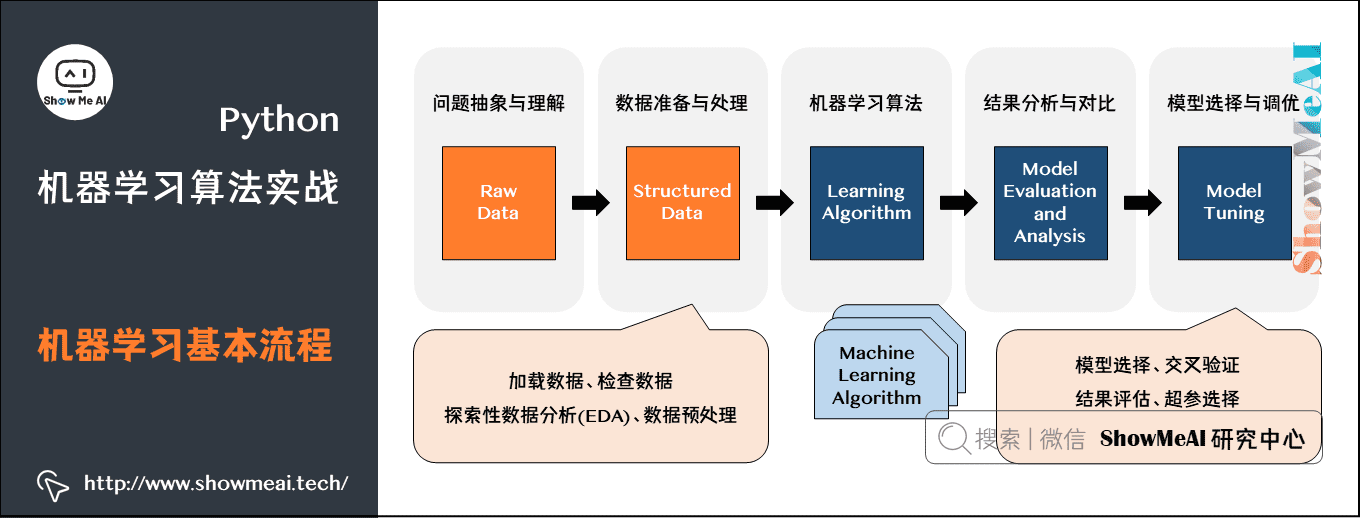• 问题抽象与理解
• 数据准备与处理(预处理、特征提取、特征工程等)
• 各种机器学习算法
• 实验结果分析与对比
• 模型选择与调优

我们会覆盖到的机器学习算法包括：KNN、朴素贝叶斯、逻辑回归、SVM、决策树、随机森林、感知机、前馈神经网络、卷积神经网络。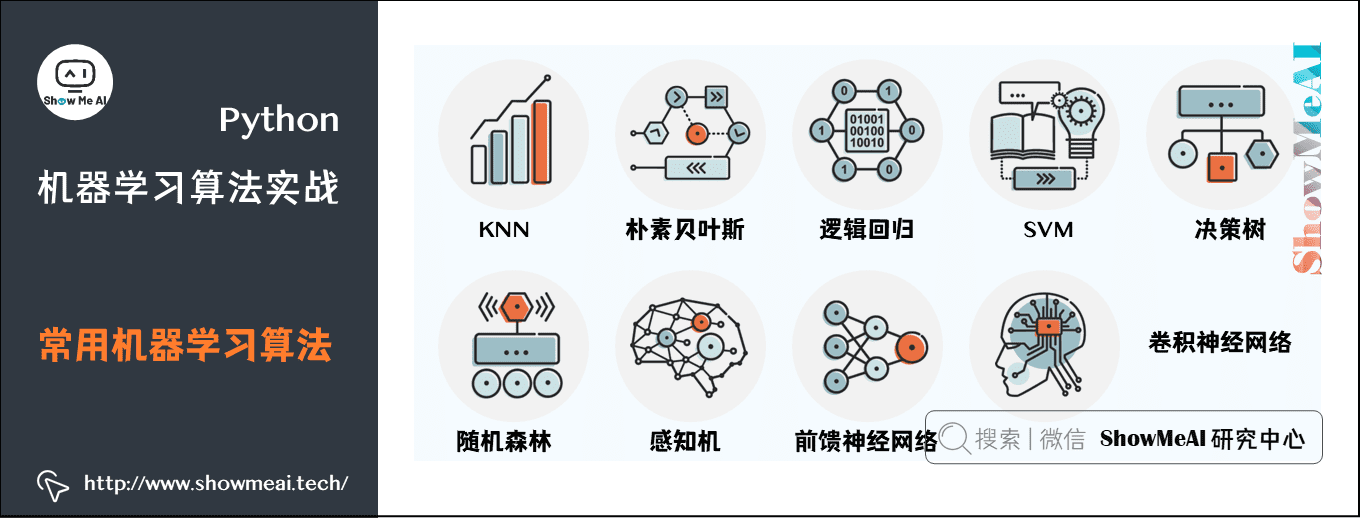# 1.环境准备

工欲善其事必先利其器，我们先安装一下必需的Python库(当然我们也推荐大家用集成环境anaconda，具体的安装与设置可以参考ShowMeAI文章 图解Python | 安装与环境设置 完成)：

可以采用pip安装，命令如下：
$pip install numpy$ pip install pillow
$pip install --upgrade scikit-learn$ pip install tensorflow # or tensorflow-gpu
$pip install keras$ pip install opencv-contrib-python
$pip install --upgrade imutils  # 2.数据集 因为本篇文章我们介绍结构化数据和非结构化数据的不同建模，我们这里用到两个数据集。 ## 2.1 iris(鸢尾花)数据集 第一个数据集是iris(鸢尾花)数据集，它是一个入门级数据集。整个数据集都是数值型的数据，是一个结构化的表格数据，每一行代表一个样本，然后每一列就是不同的属性。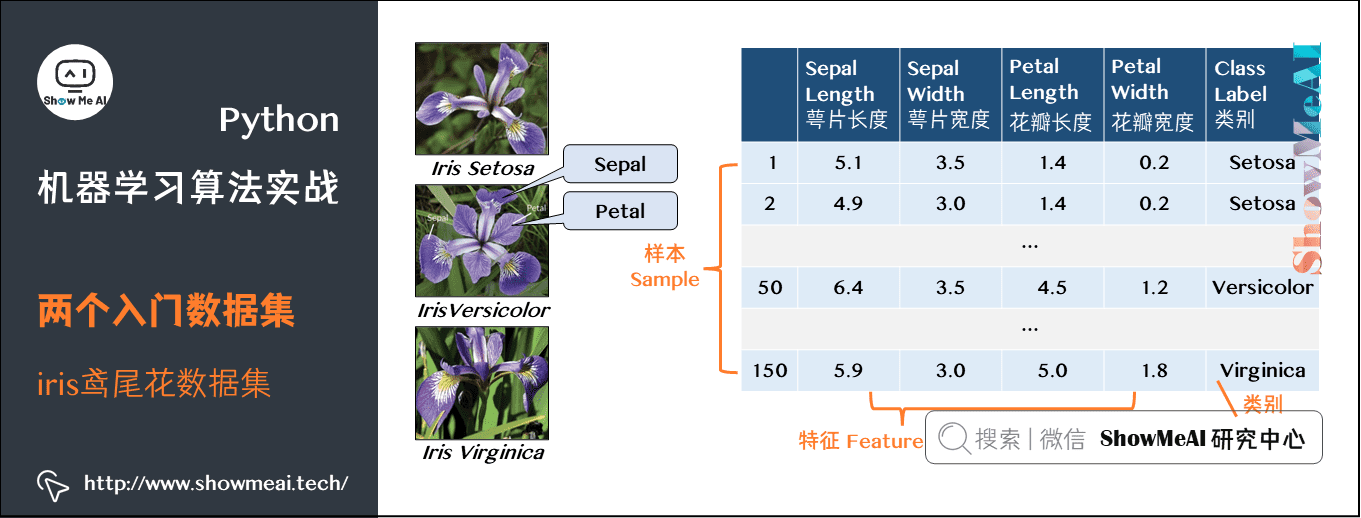这个数据集主要是收集了三种不同的鸢尾花的数据，分别为： • Iris Setosa • Iris Versicolor • Iris Virginica 对应图中最后一列Class label，然后还有四种属性，分别是： • Sepal length：萼片长度 • Sepal width：萼片宽度 • Petal length：花瓣长度 • Petal width：花瓣宽度 对于该数据集，我们的目标就是根据给定的四个属性，训练一个机器学习模型来正确分类每个样本的类别，这是一个典型的分类任务。 ## 2.2 图像数据集 第二个数据集是一个图像数据集。它包括海岸线(Coast)、森林(Forest)和高速公路(Highway)三种场景，总共是 948 张图片，我们需要构建模型完成类别的分类，每个类别的具体图片数量如下： • 海岸线(Coast)：360 • 森林(Forest)：328 • 高速公路(Highway)：260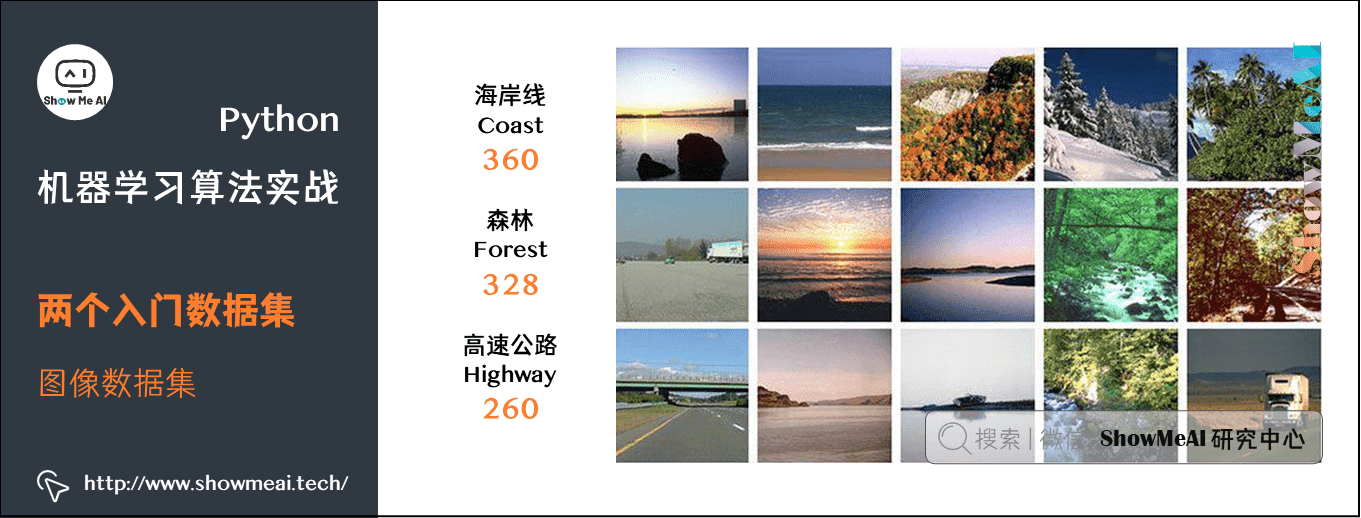# 3.机器学习应用步骤 我们在不同场景下应用机器学习算法，都有大致的步骤，比如下面是一个典型的机器学习应用流程：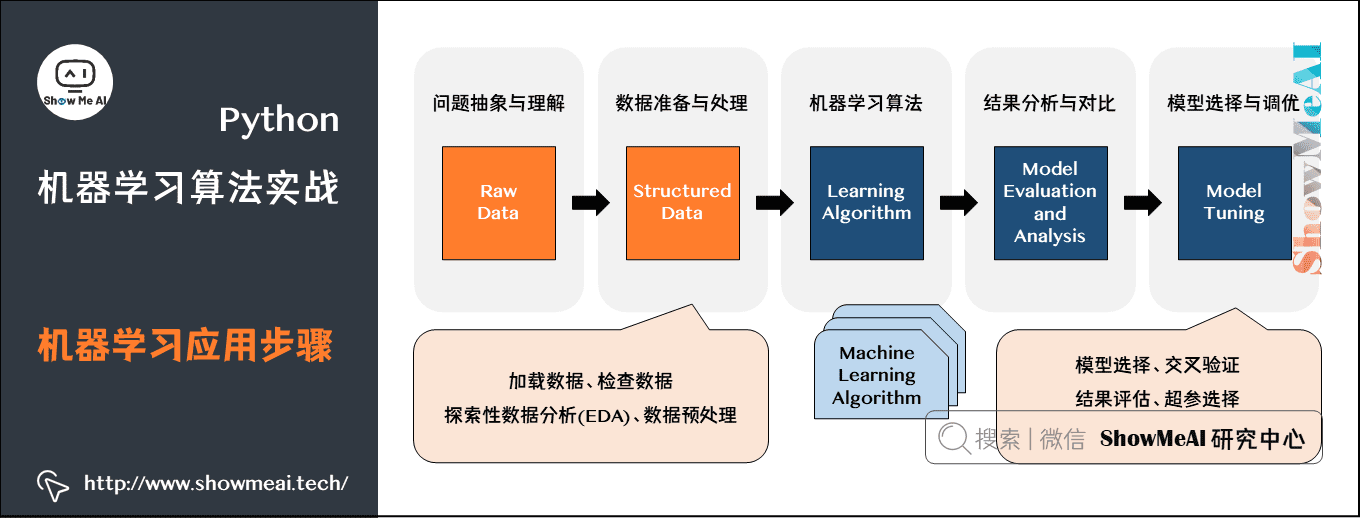当然，并不是其中的每一步都是必须的，我们也可能会调整其中某些步骤中的细节。 ## 3.1 问题抽象与理解 针对我们的问题，问一下自己： • 数据集是哪种类型？数值型，类别型还是图像？ • 模型的最终目标是什么？ • 如何定义和衡量“准确率”呢？ • 以目前自身的机器学习知识来看，哪些算法在处理这类问题上效果很好？ 前序问题比较简单，最后的问题，随着大家应用机器学习解决问题的经验积累，可以更准确快速地回答。 ## 3.2 数据准备与处理 数据准备与处理，包括数据预处理以及特征工程了。一般这一步，包括了加载数据、检查数据、探索性数据分析(EDA)、数据预处理，进而决定需要做的特征提取或者特征工程。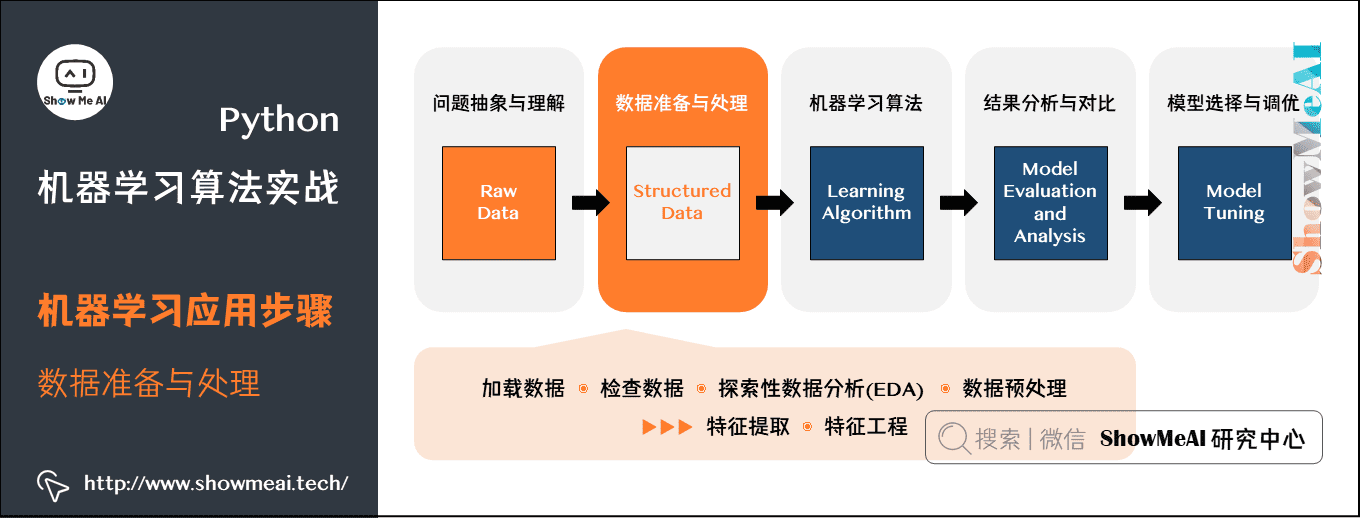特征提取是应用某种算法通过某种方式来量化数据的过程。比如，对于图像数据，我们可以采用计算直方图的方法来统计图像中像素强度的分布，通过这种方式，我们就得到描述图像颜色的特征。 特征工程是将原始输入数据转换成一个更好描述潜在问题的特征表示的过程。大家可以查看ShowMeAI机器学习专题文章 系统了解特征工程的常见方法。 ## 3.3 多模型应用 下一步可以选择各种候选机器学习算法，并应用在数据集上。我们安装的工具包内，包含很多机器学习算法，比如下述模型都可以用作分类： • 线性模型(逻辑回归、线性SVM) • 非线性模型(RBF、SVM、梯度下降分类器) • 树和基于集成的模型(决策树、随机森林) • 神经网络(多层感知机、卷积神经网络) 对于模型选择，当然很多需要依据实验效果来定，但我们也有一些先序的经验，比如： • 对于稠密型多特征的数据集，随机森林算法的效果很不错； • 逻辑回归算法可以很好处理高维度的稀疏数据； • 对于图像数据，CNNs的效果非常好。 下图为 scikit-learn工具库 官方给的一个模型选择思路参考：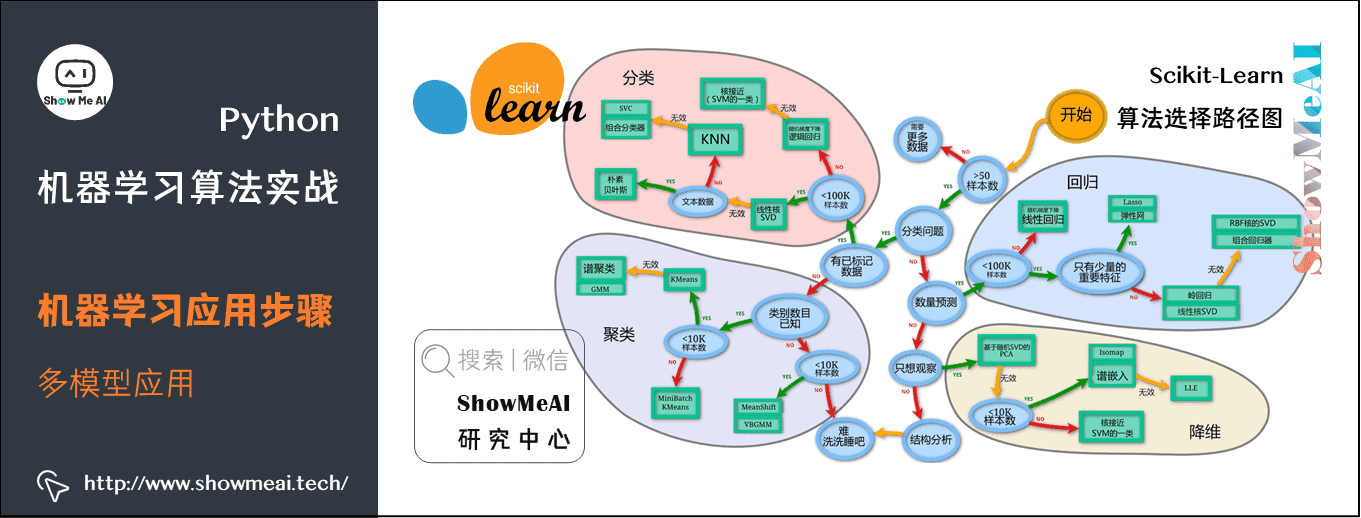# 4.构建机器学习流程并实验分析 我们构建如下的代码文件目录，包含四个代码文件和一个3scenes图像文件夹(内含三场景数据集)，iris数据集无需另外存储，直接采用scikit-learn库载入即可。 ├── 3scenes │ ├── coast [360 entries] │ ├── forest [328 entries] │ └── highway [260 entries] ├── iris_classifier.py ├── image_classifier.py ├── nn_iris.py └── basic_cnn.py  ## 4.1 结构化数据建模 首先实现iris_classifier，我们这里直接使用sklearn的机器学习算法来对iris数据集进行分类。 # 导入需要的库 from sklearn.neighbors import KNeighborsClassifier from sklearn.naive_bayes import GaussianNB from sklearn.linear_model import LogisticRegression from sklearn.svm import SVC from sklearn.tree import DecisionTreeClassifier from sklearn.ensemble import RandomForestClassifier from sklearn.neural_network import MLPClassifier from sklearn.model_selection import train_test_split from sklearn.metrics import classification_report from sklearn.datasets import load_iris import argparse # 设置参数 ap = argparse.ArgumentParser() ap.add_argument("-m", "--model", type=str, default="knn", help="type of python machine learning model to use") args = vars(ap.parse_args()) # 定义一个保存模型的字典，根据 key 来选择加载哪个模型 models = { "knn": KNeighborsClassifier(n_neighbors=1), "naive_bayes": GaussianNB(), "logit": LogisticRegression(solver="lbfgs", multi_class="auto"), "svm": SVC(kernel="rbf", gamma="auto"), "decision_tree": DecisionTreeClassifier(), "random_forest": RandomForestClassifier(n_estimators=100), "mlp": MLPClassifier() }  其中，models从前往后依次包括这些算法：KNN、朴素贝叶斯、逻辑回归、SVM、决策树、随机森林、感知机等。 我们直接调用sklearn中相应的函数来实现对应的算法即可，这里直接用一个models的字典来保存不同模型的初始化，然后根据参数--model来调用对应的模型，比如命令输入python iris_classifier.py --model knn就是调用knn算法模型。 接着就是载入数据部分： print("加载数据中...") dataset = load_iris() trainX, testX, trainY, testY = train_test_split(dataset.data, dataset.target, random_state=3, test_size=0.2)  这里直接调用sklearn.datasets中的load_iris()载入数据，然后采用train_test_split来划分训练集和数据集，这里是80%数据作为训练集，20%作为测试集。 最后就是训练模型和预测部分： # 训练模型 print("应用 '{}' 模型建模...".format(args["model"])) model = models[args["model"]] model.fit(trainX, trainY) # 预测并输出一份分类结果报告 print("评估模型效果...") predictions = model.predict(testX) print(classification_report(testY, predictions, target_names=dataset.target_names))  ## 4.2 图像数据建模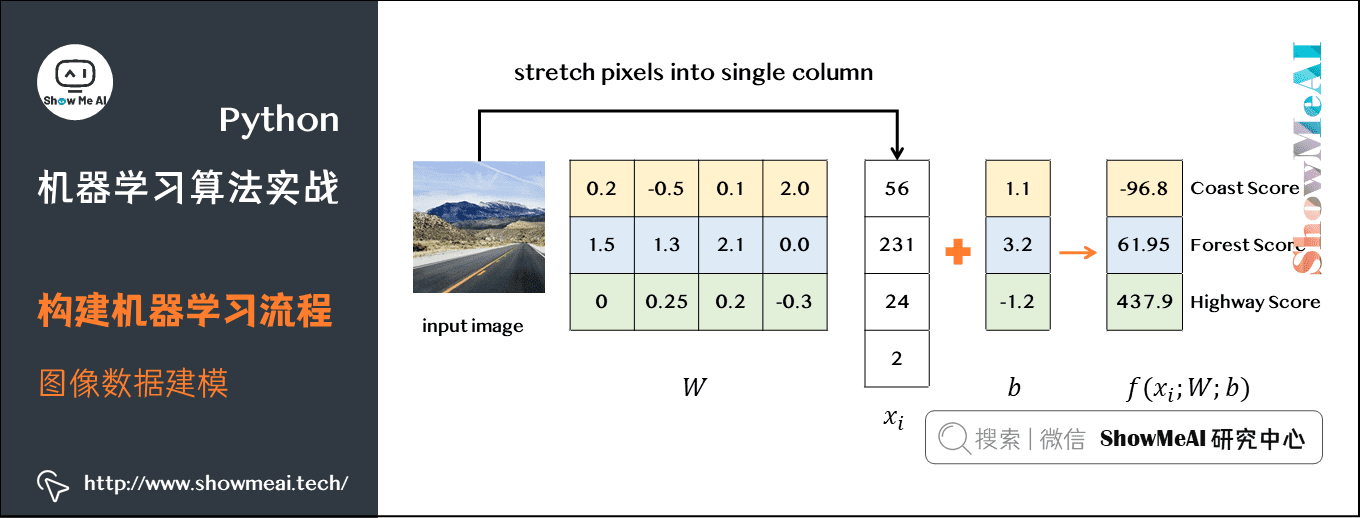类似的过程对三场景图像数据集构建代码image_classifier.py # 导入工具库 from sklearn.preprocessing import LabelEncoder from PIL import Image from imutils import paths import numpy as np import os  其中LabelEncoder是为了将标签从字符串编码为整型，然后其余几项都是处理图像相关。 对于图像数据，如果直接采用原始像素信息输入模型中，大部分的机器学习算法效果都很不理想，所以这里采用特征提取方法，主要是统计图像颜色通道的均值和标准差信息，总共是RGB3个通道，每个通道各计算均值和标准差，然后结合在一起，得到一个六维的特征，函数如下所示： def extract_color_stats(image): ''' 将图片分成 RGB 三通道，然后分别计算每个通道的均值和标准差，然后返回 :param image: :return: ''' (R, G, B) = image.split() features = [np.mean(R), np.mean(G), np.mean(B), np.std(R), np.std(G), np.std(B)] return features  然后同样会定义一个models字典，代码一样，这里就不贴出来了，然后图像载入部分的代码如下： # 加载数据并提取特征 print("抽取图像特征中...") imagePaths = paths.list_images(args['dataset']) data = [] labels = [] # 循环遍历所有的图片数据 for imagePath in imagePaths: # 加载图片，然后计算图片的颜色通道统计信息 image = Image.open(imagePath) features = extract_color_stats(image) data.append(features) # 保存图片的标签信息 label = imagePath.split(os.path.sep)[-2] labels.append(label) # 对标签进行编码，从字符串变为整数类型 le = LabelEncoder() labels = le.fit_transform(labels) # 进行训练集和测试集的划分，80%数据作为训练集，其余20%作为测试集 trainX, testX, trainY, testY = train_test_split(data, labels, test_size=0.2)  上述代码就完成加载图片的路径信息，然后依次遍历，读取图片，提取特征，提取标签信息，保存特征和标签信息，接着编码标签，然后就是划分训练集和测试集。 接着是相同的训练模型和预测的代码，和前面的分类器一样。完整版代码如下： # 导入工具库 from sklearn.neighbors import KNeighborsClassifier from sklearn.naive_bayes import GaussianNB from sklearn.linear_model import LogisticRegression from sklearn.svm import SVC from sklearn.tree import DecisionTreeClassifier from sklearn.ensemble import RandomForestClassifier from sklearn.neural_network import MLPClassifier from sklearn.preprocessing import LabelEncoder from sklearn.model_selection import train_test_split from sklearn.metrics import classification_report from PIL import Image from imutils import paths import numpy as np import argparse import os # 抽取图像特征 def extract_color_stats(image): ''' 将图片分成 RGB 三通道，然后分别计算每个通道的均值和标准差，然后返回 :param image: :return: ''' (R, G, B) = image.split() features = [np.mean(R), np.mean(G), np.mean(B), np.std(R), np.std(G), np.std(B)] return features # 设置参数 ap = argparse.ArgumentParser() ap.add_argument("-d", "--dataset", type=str, default="3scenes", help="path to directory containing the '3scenes' dataset") ap.add_argument("-m", "--model", type=str, default="knn", help="type of python machine learning model to use") args = vars(ap.parse_args()) # 定义一个保存模型的字典，根据 key 来选择加载哪个模型 models = { "knn": KNeighborsClassifier(n_neighbors=1), "naive_bayes": GaussianNB(), "logit": LogisticRegression(solver="lbfgs", multi_class="auto"), "svm": SVC(kernel="rbf", gamma="auto"), "decision_tree": DecisionTreeClassifier(), "random_forest": RandomForestClassifier(n_estimators=100), "mlp": MLPClassifier() } # 加载数据并提取特征 print("抽取图像特征中...") imagePaths = paths.list_images(args['dataset']) data = [] labels = [] # 循环遍历所有的图片数据 for imagePath in imagePaths: # 加载图片，然后计算图片的颜色通道统计信息 image = Image.open(imagePath) features = extract_color_stats(image) data.append(features) # 保存图片的标签信息 label = imagePath.split(os.path.sep)[-2] labels.append(label) # 对标签进行编码，从字符串变为整数类型 le = LabelEncoder() labels = le.fit_transform(labels) # 进行训练集和测试集的划分，80%数据作为训练集，其余20%作为测试集 trainX, testX, trainY, testY = train_test_split(data, labels, random_state=3, test_size=0.2) # print('trainX numbers={}, testX numbers={}'.format(len(trainX), len(testX))) # 训练模型 print("[应用 '{}' 模型建模".format(args["model"])) model = models[args["model"]] model.fit(trainX, trainY) # 预测并输出分类结果报告 print("模型评估") predictions = model.predict(testX) print(classification_report(testY, predictions, target_names=le.classes_))  完成这两份代码后，我们就可以开始运行下代码，对比不同算法在两个数据集上的性能。 ## 4.3 不同模型建模对比 ### (1) KNN K-Nearest Neighbors分类器最简单的分类算法之一。该算法依赖于特征向量之间的距离。简单地说，KNN算法通过在k个最接近的样本中最多的类别来对未知数据点进行分类。关于KNN的详细讲解可以阅读ShowMeAI的文章 图解机器学习 | KNN算法及其应用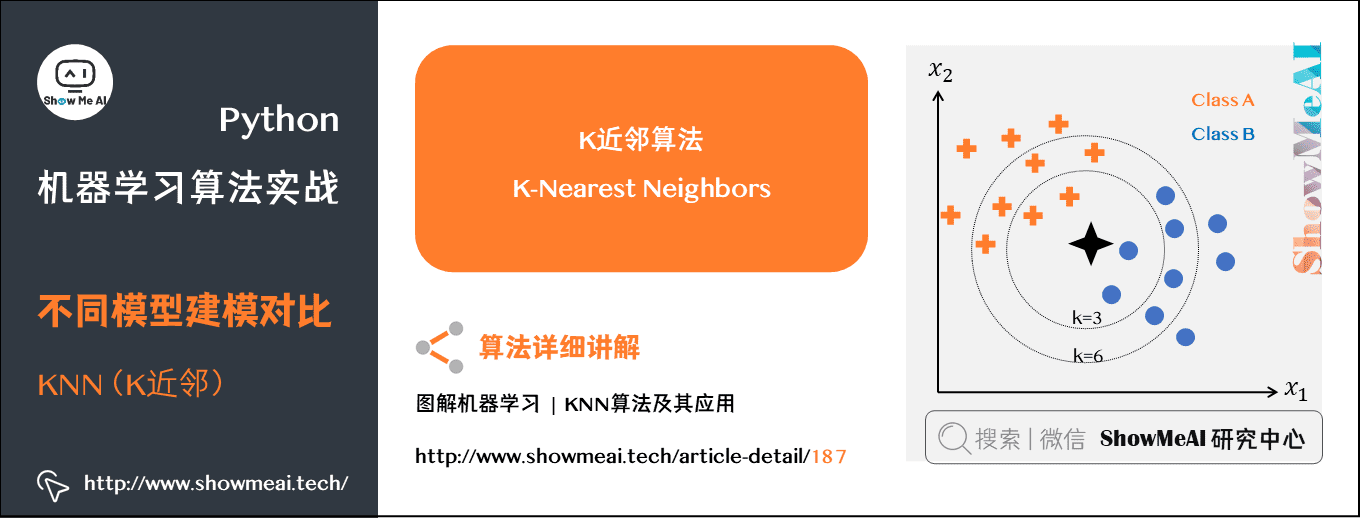这里我们先运行下image_classifier.py，调用默认的模型knn，看下KNNiris数据集上的实验结果，如下所示： $ !python iris_classifier.py --model knn
加载数据中...
应用 'knn' 模型建模...
评估模型效果...
precision    recall  f1-score   support

setosa       1.00      1.00      1.00        10
versicolor       0.90      0.90      0.90        10
virginica       0.90      0.90      0.90        10

accuracy                           0.93        30
macro avg       0.93      0.93      0.93        30
weighted avg       0.93      0.93      0.93        30


其中主要是给出了对每个类别的精确率、召回率、F1以及该类别测试集数量，即分别对应precision、recall、f1-score、support。根据最后一行第一列，可以看到KNN取得93%的准确率。

接着是在三场景图片数据集上的实验结果：

$!python image_classifier.py --model knn 抽取图像特征中... 应用 'knn' 模型建模... 评估模型效果... precision recall f1-score support coast 0.84 0.68 0.75 105 forest 0.78 0.77 0.77 78 highway 0.56 0.78 0.65 54 micro avg 0.73 0.73 0.73 237 macro avg 0.72 0.74 0.72 237 weighted avg 0.75 0.73 0.73 237  这里KNN取得75%的准确率。 ps：实际上，运行这个算法，不同次数会有不同的结果，其主要原因是因为在划分训练集和测试集的时候，代码没有设置参数random_state，这导致每次运行划分的训练集和测试集的图片都是不同的，所以运行结果也会不相同！ ### (2) 朴素贝叶斯 接着是朴素贝叶斯算法，关于朴素贝叶斯算法的详细讲解可以阅读ShowMeAI的文章 图解机器学习 | 朴素贝叶斯算法详解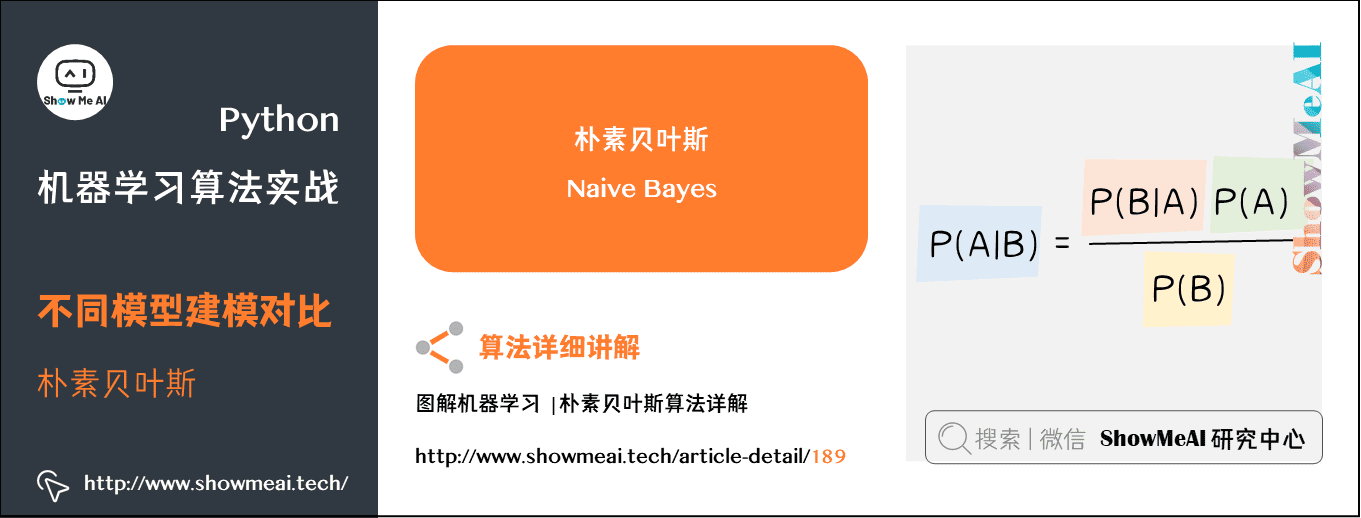分别测试两个数据集，结果如下： $ !python iris_classifier.py --model naive_bayes
加载数据中...
应用 'naive_bayes' 模型建模...
评估模型效果...
precision    recall  f1-score   support

setosa       1.00      1.00      1.00        15
versicolor       1.00      0.92      0.96        12
virginica       0.92      1.00      0.96        11

micro avg       0.97      0.97      0.97        38
macro avg       0.97      0.97      0.97        38
weighted avg       0.98      0.97      0.97        38

$!python image_classifier.py --model naive_bayes 抽取图像特征中... 应用 'naive_bayes' 模型建模... 评估模型效果... precision recall f1-score support coast 0.69 0.40 0.50 88 forest 0.68 0.82 0.74 84 highway 0.61 0.78 0.68 65 micro avg 0.65 0.65 0.65 237 macro avg 0.66 0.67 0.64 237 weighted avg 0.66 0.65 0.64 237  同样，朴素贝叶斯在iris上有98%的准确率，但是在图像数据集上仅有66%的准确率。 那么，我们是否可以说明KNN算法比朴素贝叶斯好呢？当然是不可以的，上述结果只能说明在三场景图像数据集上，KNN算法优于朴素贝叶斯算法。 实际上，每种算法都有各自的优缺点和适用场景，不能一概而论地说某种算法任何时候都优于另一种算法，这需要具体问题具体分析。 ### (3) 逻辑回归 接着是逻辑回归算法，关于逻辑回归算法的详细讲解可以阅读ShowMeAI的文章 图解机器学习 | 逻辑回归算法详解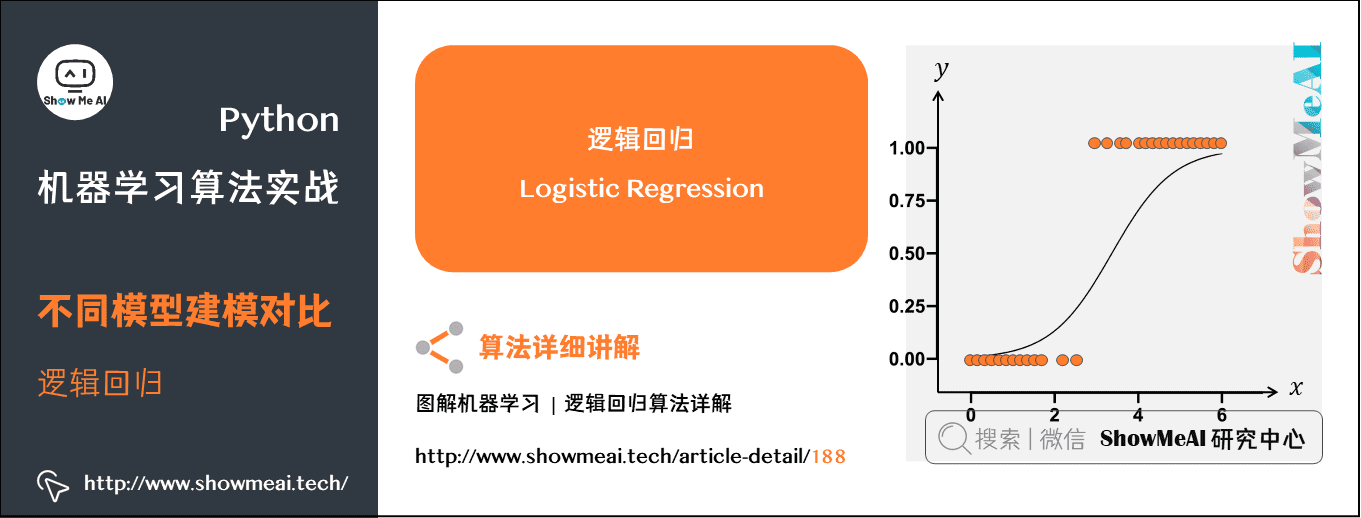分别测试两个数据集，结果如下： $ !python iris_classifier.py --model logit
加载数据中...
应用 'logit' 模型建模...
评估模型效果...
precision    recall  f1-score   support

setosa       1.00      1.00      1.00        15
versicolor       1.00      0.92      0.96        12
virginica       0.92      1.00      0.96        11

micro avg       0.97      0.97      0.97        38
macro avg       0.97      0.97      0.97        38
weighted avg       0.98      0.97      0.97        38

$!python image_classifier.py --model logit 抽取图像特征中... 应用 'logit' 模型建模... 评估模型效果... precision recall f1-score support coast 0.67 0.67 0.67 92 forest 0.79 0.82 0.80 82 highway 0.61 0.57 0.59 63 micro avg 0.70 0.70 0.70 237 macro avg 0.69 0.69 0.69 237 weighted avg 0.69 0.70 0.69 237  同样，逻辑回归在 iris 上有 98% 的准确率，但是在图像数据集上仅有 69% 的准确率 ### (4) 支持向量机 SVM 接着是SVM算法，关于SVM算法的详细讲解可以阅读ShowMeAI的文章 图解机器学习 | 支持向量机模型详解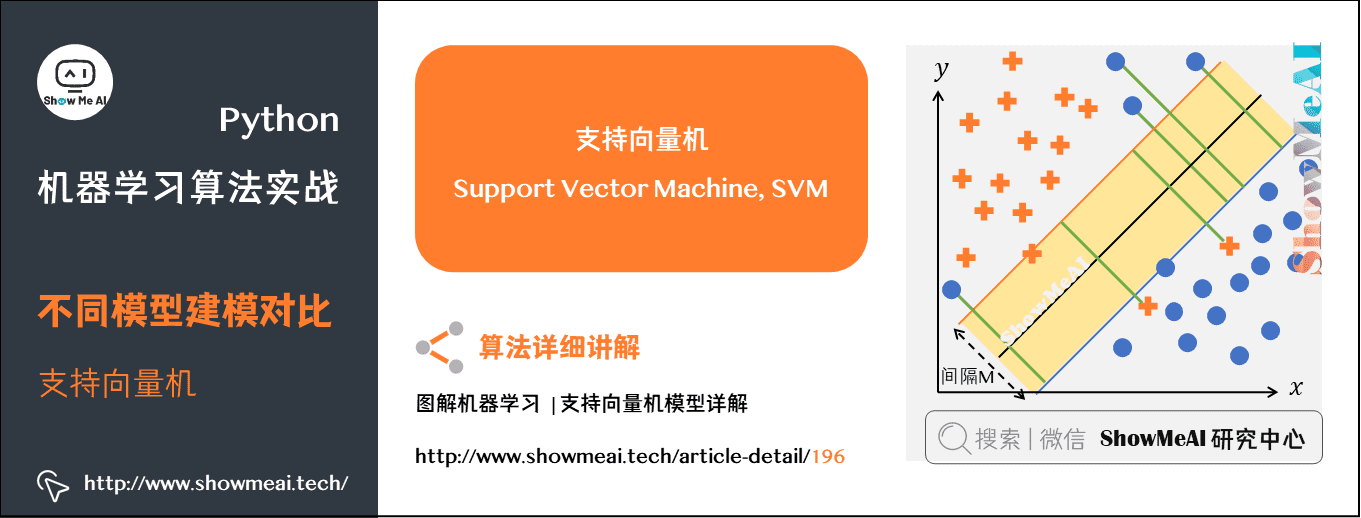分别测试两个数据集，结果如下： $ !python iris_classifier.py --model svm
加载数据中...
应用 'svm' 模型建模...
评估模型效果...
precision    recall  f1-score   support

setosa       1.00      1.00      1.00        15
versicolor       1.00      0.92      0.96        12
virginica       0.92      1.00      0.96        11

micro avg       0.97      0.97      0.97        38
macro avg       0.97      0.97      0.97        38
weighted avg       0.98      0.97      0.97        38

$!python image_classifier.py --model svm 抽取图像特征中... 应用 'svm' 模型建模... 评估模型效果... precision recall f1-score support coast 0.84 0.76 0.80 92 forest 0.86 0.93 0.89 84 highway 0.78 0.80 0.79 61 micro avg 0.83 0.83 0.83 237 macro avg 0.83 0.83 0.83 237  同样，SVM在iris上有98%的准确率，但是在图像数据集上仅有83%的准确率。 ### (5) 决策树 接着是决策树算法，关于决策树算法的详细讲解可以阅读ShowMeAI的文章 图解机器学习 | 决策树模型详解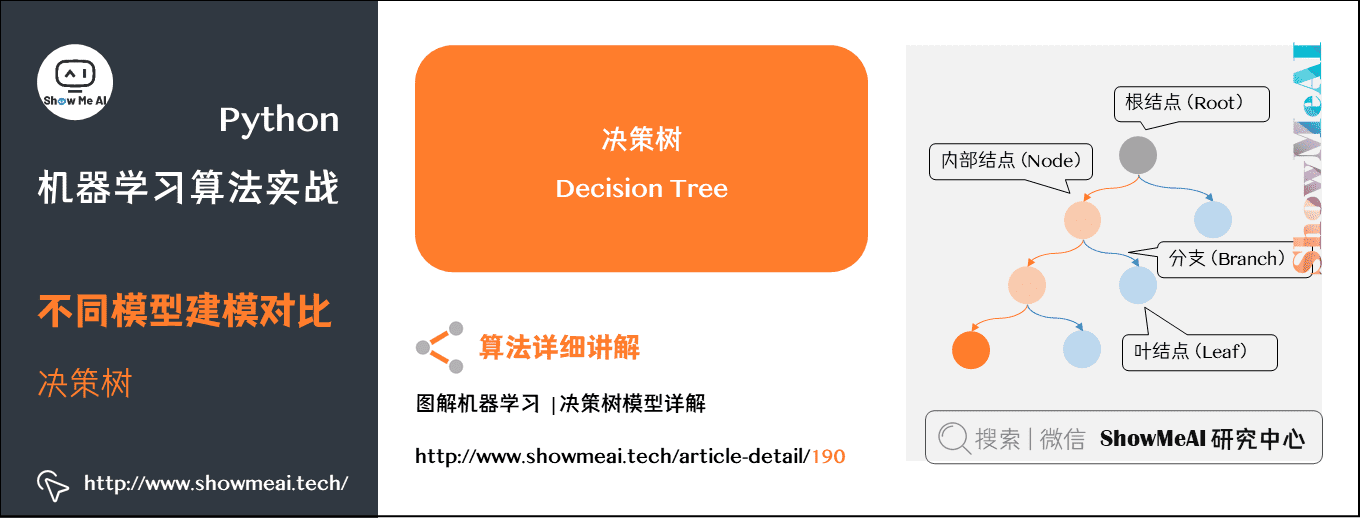分别测试两个数据集，结果如下： $ !python iris_classifier.py --model decision_tree
加载数据中...
应用 'decision_tree' 模型建模...
评估模型效果...
precision    recall  f1-score   support

setosa       1.00      1.00      1.00        15
versicolor       0.92      0.92      0.92        12
virginica       0.91      0.91      0.91        11

micro avg       0.95      0.95      0.95        38
macro avg       0.94      0.94      0.94        38
weighted avg       0.95      0.95      0.95        38

$!python image_classifier.py --model decision_tree 抽取图像特征中... 应用 'decision_tree' 模型建模... 评估模型效果... precision recall f1-score support coast 0.71 0.74 0.72 85 forest 0.76 0.80 0.78 83 highway 0.77 0.68 0.72 69 micro avg 0.74 0.74 0.74 237 macro avg 0.75 0.74 0.74 237 weighted avg 0.74 0.74 0.74 237  同样，决策树在iris上有98%的准确率，但是在图像数据集上仅有74%的准确率。 ### (6) 随机森林 接着是随机森林算法，关于随机森林算法的详细讲解可以阅读ShowMeAI的文章 图解机器学习 | 随机森林分类模型详解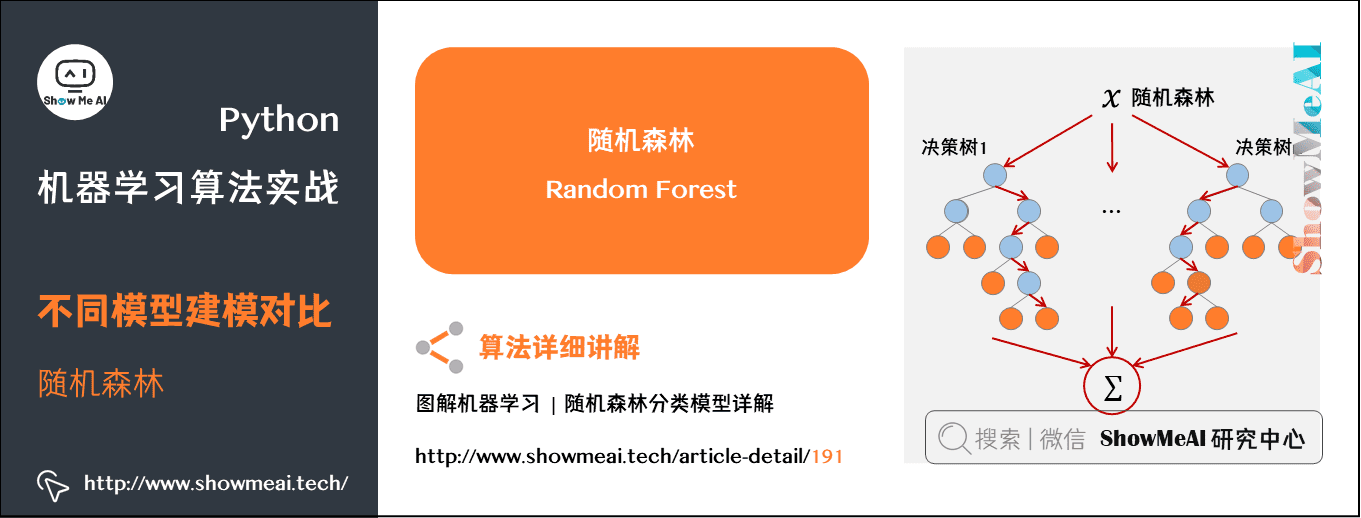分别测试两个数据集，结果如下： $ !python iris_classifier.py --model random_forest
加载数据中...
应用 'random_forest' 模型建模...
评估模型效果...
precision    recall  f1-score   support

setosa       1.00      1.00      1.00        15
versicolor       1.00      0.83      0.91        12
virginica       0.85      1.00      0.92        11

micro avg       0.95      0.95      0.95        38
macro avg       0.95      0.94      0.94        38
weighted avg       0.96      0.95      0.95        38

$!python image_classifier.py --model random_forest 加载数据中... 应用 'random_forest' 模型建模... 评估模型效果... precision recall f1-score support coast 0.80 0.83 0.81 84 forest 0.92 0.84 0.88 90 highway 0.77 0.81 0.79 63 micro avg 0.83 0.83 0.83 237 macro avg 0.83 0.83 0.83 237 weighted avg 0.84 0.83 0.83 237  同样，随机森林在iris上有96%的准确率，但是在图像数据集上仅有84%的准确率。 注意了，一般如果决策树算法的效果还不错的话，随机森林算法应该也会取得不错甚至更好的结果，这是因为随机森林实际上就是多棵决策树通过集成学习方法组合在一起进行分类预测。 ### (7) 多层感知机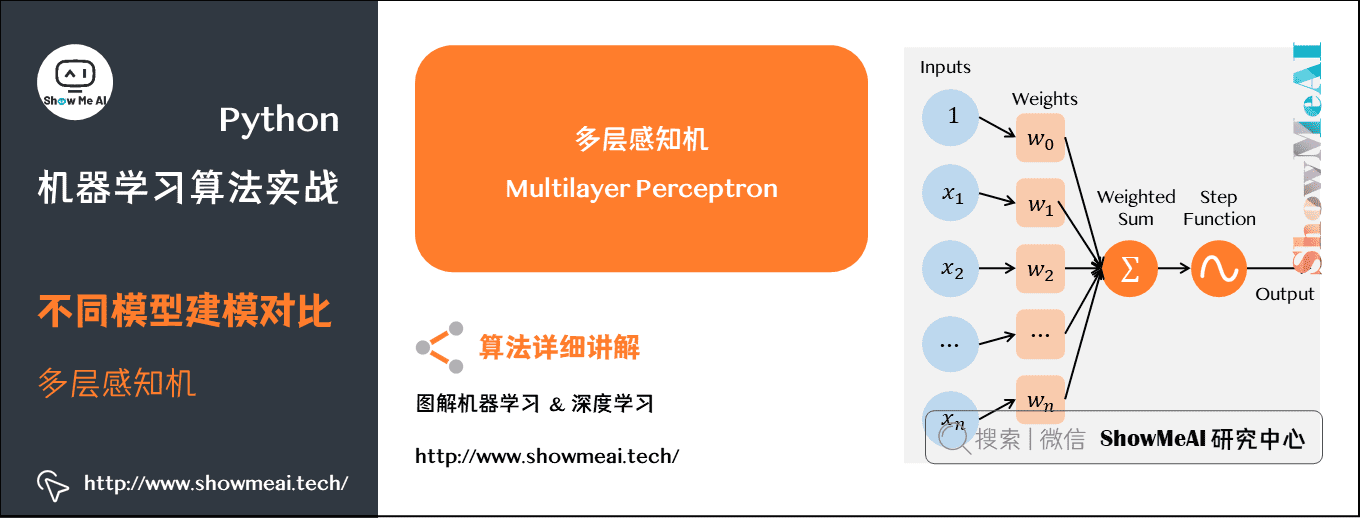最后是多层感知机算法，分别测试两个数据集，结果如下： $ !python iris_classifier.py --model mlp
加载数据中...
应用 'mlp' 模型建模...
评估模型效果...
precision    recall  f1-score   support

setosa       1.00      1.00      1.00        15
versicolor       1.00      0.92      0.96        12
virginica       0.92      1.00      0.96        11

micro avg       0.97      0.97      0.97        38
macro avg       0.97      0.97      0.97        38
weighted avg       0.98      0.97      0.97        38

$!python image_classifier.py --model mlp 抽取图像特征中... 应用 'mlp' 模型建模... 评估模型效果... precision recall f1-score support coast 0.72 0.91 0.80 86 forest 0.92 0.89 0.90 79 highway 0.79 0.58 0.67 72 micro avg 0.80 0.80 0.80 237 macro avg 0.81 0.79 0.79 237 weighted avg 0.81 0.80 0.80 237  同样，多层感知机在 iris 上有 98% 的准确率，但是在图像数据集上仅有 81% 的准确率. ### (8) 神经网络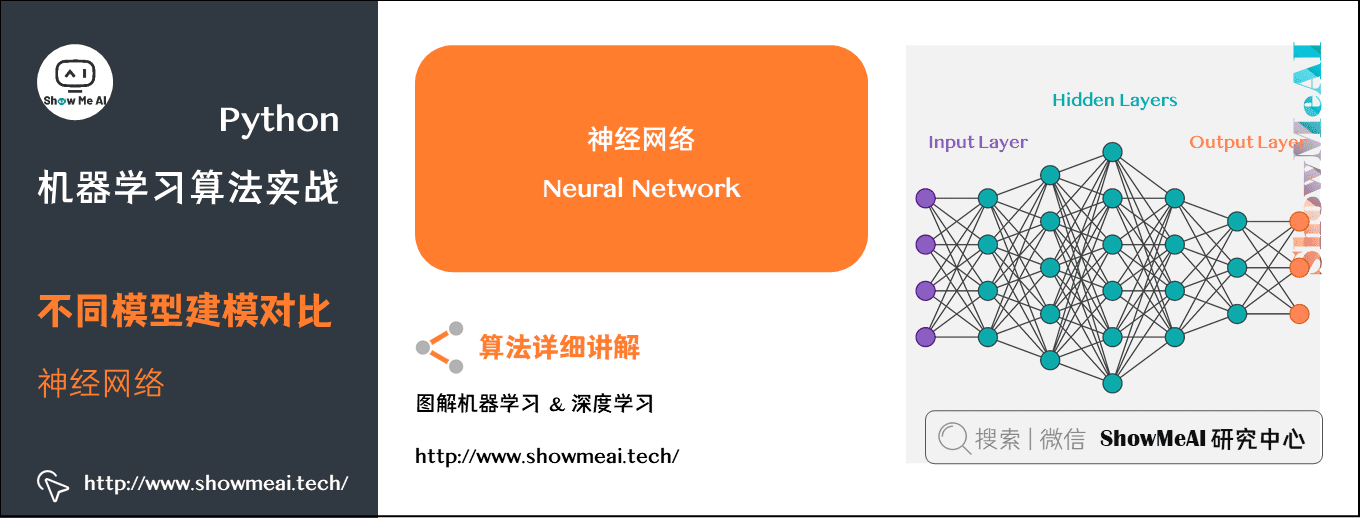最后是实现深度学习的算法，也就是nn_iris.pybasic_cnn.py这两份代码。 首先是nn_iris.py的实现，同样首先是导入库和数据的处理： # 导入工具库 from keras.models import Sequential from keras.layers.core import Dense from keras.optimizers import SGD from sklearn.preprocessing import LabelBinarizer from sklearn.model_selection import train_test_split from sklearn.metrics import classification_report from sklearn.datasets import load_iris # 载入 Iris 数据集，然后进行训练集和测试集的划分，80%数据作为训练集，其余20%作为测试集 print("加载数据中...") dataset = load_iris() (trainX, testX, trainY, testY) = train_test_split(dataset.data, dataset.target, test_size=0.2) # 将标签进行独热向量编码 lb = LabelBinarizer() trainY = lb.fit_transform(trainY) testY = lb.transform(testY)  我们采用Keras来实现神经网络，然后这里需要将标签进行one-hot编码，即独热向量编码。 接着就是搭建网络模型的结构和训练、预测代码： # 利用 Keras 定义网络模型 model = Sequential() model.add(Dense(3, input_shape=(4,), activation="sigmoid")) model.add(Dense(3, activation="sigmoid")) model.add(Dense(3, activation="softmax")) # 采用梯度下降训练模型 print('训练网络中...') opt = SGD(lr=0.1, momentum=0.9, decay=0.1 / 250) model.compile(loss='categorical_crossentropy', optimizer=opt, metrics=["accuracy"]) H = model.fit(trainX, trainY, validation_data=(testX, testY), epochs=250, batch_size=16) # 预测 print('评估模型效果') predictions = model.predict(testX, batch_size=16) print(classification_report(testY.argmax(axis=1), predictions.argmax(axis=1), target_names=dataset.target_names))  上述代码构建了3层全连接层的神经网络，前两层采用Sigmoid激活函数，然后最后一层是输出层，所以采用softmax将输出变成概率值。优化算法选择的随机梯度下降SGD，损失函数是categorical_crossentropy，迭代次数是250次，每一批次的数据量batch_size是16。 完整版代码如下： # 加载工具库 from keras.models import Sequential from keras.layers.core import Dense from keras.optimizers import SGD from sklearn.preprocessing import LabelBinarizer from sklearn.model_selection import train_test_split from sklearn.metrics import classification_report from sklearn.datasets import load_iris # 载入 Iris 数据集，然后进行训练集和测试集的划分，80%数据作为训练集，其余20%作为测试集 print("加载数据中...") dataset = load_iris() (trainX, testX, trainY, testY) = train_test_split(dataset.data, dataset.target, test_size=0.2) # 将标签进行独热向量编码 lb = LabelBinarizer() trainY = lb.fit_transform(trainY) testY = lb.transform(testY) # 利用 Keras 定义网络模型 model = Sequential() model.add(Dense(3, input_shape=(4,), activation="sigmoid")) model.add(Dense(3, activation="sigmoid")) model.add(Dense(3, activation="softmax")) # 采用梯度下降训练模型 print('训练网络中...') opt = SGD(lr=0.1, momentum=0.9, decay=0.1 / 250) model.compile(loss='categorical_crossentropy', optimizer=opt, metrics=["accuracy"]) H = model.fit(trainX, trainY, validation_data=(testX, testY), epochs=250, batch_size=16) # 预测 print('评估模型效果...') predictions = model.predict(testX, batch_size=16) print(classification_report(testY.argmax(axis=1), predictions.argmax(axis=1), target_names=dataset.target_names))  直接运行命令python nn_iris.py，输出的结果如下： $ python nn_iris.py
Using TensorFlow backend.
加载数据中...
训练网络中...
Train on 112 samples, validate on 38 samples
Epoch 1/250
2022-02-08 10:28:19.104933: I tensorflow/core/platform/cpu_feature_guard.cc:141] Your CPU supports instructions that this TensorFlow binary was not compiled to use: AVX2 AVX512F FMA
112/112 [==============================] - 0s 2ms/step - loss: 1.1454 - acc: 0.3214 - val_loss: 1.1867 - val_acc: 0.2368
Epoch 2/250
112/112 [==============================] - 0s 48us/step - loss: 1.0828 - acc: 0.3929 - val_loss: 1.2132 - val_acc: 0.5000
Epoch 3/250
112/112 [==============================] - 0s 47us/step - loss: 1.0491 - acc: 0.5268 - val_loss: 1.0593 - val_acc: 0.4737
...
Epoch 248/250
112/112 [==============================] - 0s 46us/step - loss: 0.1319 - acc: 0.9554 - val_loss: 0.0407 - val_acc: 1.0000
Epoch 249/250
112/112 [==============================] - 0s 46us/step - loss: 0.1024 - acc: 0.9643 - val_loss: 0.1595 - val_acc: 0.8947
Epoch 250/250
112/112 [==============================] - 0s 47us/step - loss: 0.0795 - acc: 0.9821 - val_loss: 0.0335 - val_acc: 1.0000
评估模型效果...
precision    recall  f1-score   support

setosa       1.00      1.00      1.00         9
versicolor       1.00      1.00      1.00        10
virginica       1.00      1.00      1.00        19

avg / total       1.00      1.00      1.00        38


这里得到的是100%的准确率。

### (9) CNN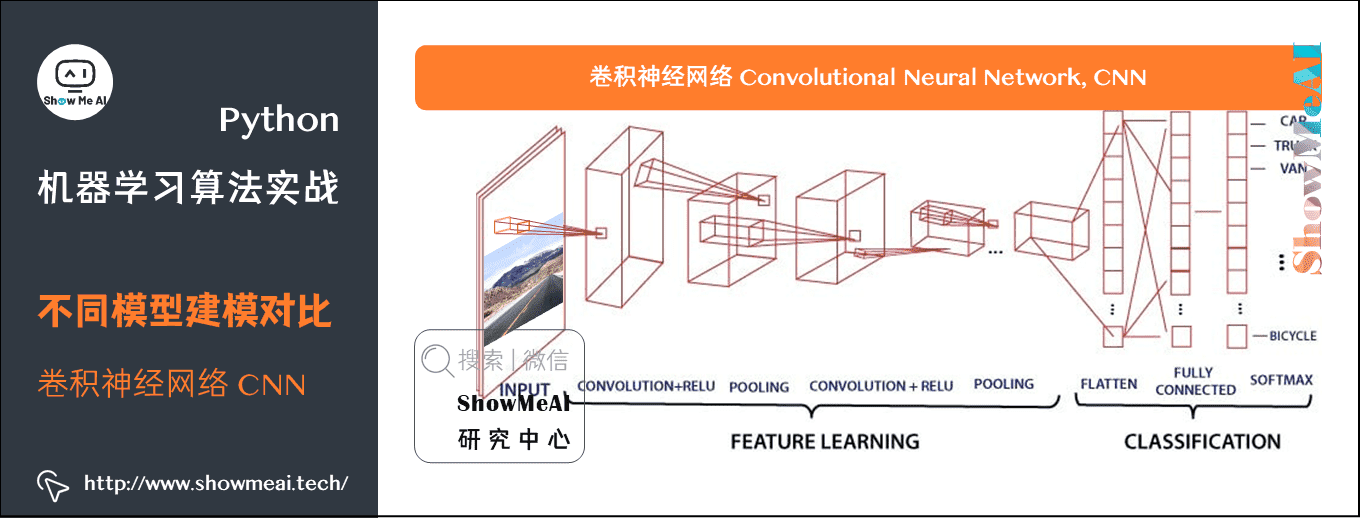最后我们要应用卷积神经网络，我们实现一下basic_cnn.py代码。

同样首先是导入必须的库函数：

# 导入工具库
from keras.models import Sequential
from keras.layers.convolutional import Conv2D
from keras.layers.convolutional import MaxPooling2D
from keras.layers.core import Activation
from keras.layers.core import Flatten
from keras.layers.core import Dense
from keras.optimizers import Adam
from sklearn.preprocessing import LabelBinarizer
from sklearn.model_selection import train_test_split
from sklearn.metrics import classification_report
from PIL import Image
from imutils import paths
import numpy as np
import argparse
import os

# 配置参数
ap = argparse.ArgumentParser()
ap.add_argument("-d", "--dataset", type=str, default="3scenes",
help="path to directory containing the '3scenes' dataset")
args = vars(ap.parse_args())



同样是要导入Keras来建立CNN的网络模型，另外因为是处理图像数据，所以PILimutils也是要导入的。

然后是加载数据和划分训练集和测试集，对于加载数据，这里直接采用原始图像像素数据，只需要对图像数据做统一尺寸的调整，这里是统一调整为32×32，并做归一化到[0,1]的范围。

# 加载数据并提取特征
print("抽取图像特征中...")
imagePaths = paths.list_images(args['dataset'])
data = []
labels = []

# 循环遍历所有的图片数据
for imagePath in imagePaths:
# 加载图片，然后调整成 32×32 大小，并做归一化到 [0,1]
image = Image.open(imagePath)
image = np.array(image.resize((32, 32))) / 255.0
data.append(image)

# 保存图片的标签信息
label = imagePath.split(os.path.sep)[-2]
labels.append(label)

# 对标签编码，从字符串变为整型
lb = LabelBinarizer()
labels = lb.fit_transform(labels)

# 划分训练集和测试集
(trainX, testX, trainY, testY) = train_test_split(np.array(data), np.array(labels), test_size=0.25)


接着定义了一个4层的CNN网络结构，包含3层卷积层和最后一层输出层，优化算法采用的是Adam而不是SGD。代码如下所示：

# 定义 CNN 网络模型结构
model = Sequential()
model.add(Conv2D(8, (3, 3), padding="same", input_shape=(32, 32, 3)))
model.add(MaxPooling2D(pool_size=(2, 2), strides=(2, 2)))
model.add(MaxPooling2D(pool_size=(2, 2), strides=(2, 2)))
model.add(MaxPooling2D(pool_size=(2, 2), strides=(2, 2)))

# 训练模型
print("训练网络中...")
opt = Adam(lr=1e-3, decay=1e-3 / 50)
model.compile(loss="categorical_crossentropy", optimizer=opt, metrics=["accuracy"])
H = model.fit(trainX, trainY, validation_data=(testX, testY),
epochs=50, batch_size=32)

# 预测
print("评估模型效果...")
predictions = model.predict(testX, batch_size=32)
print(classification_report(testY.argmax(axis=1),
predictions.argmax(axis=1), target_names=lb.classes_))


运行命令python basic_cnn.py，输出结果如下：

\$ python basic_cnn.py
Using TensorFlow backend.
加载图像数据...
训练网络中...
Train on 711 samples, validate on 237 samples
Epoch 1/50
711/711 [==============================] - 0s 629us/step - loss: 1.0647 - acc: 0.4726 - val_loss: 0.9920 - val_acc: 0.5359
Epoch 2/50
711/711 [==============================] - 0s 313us/step - loss: 0.9200 - acc: 0.6188 - val_loss: 0.7778 - val_acc: 0.6624
Epoch 3/50
711/711 [==============================] - 0s 308us/step - loss: 0.6775 - acc: 0.7229 - val_loss: 0.5310 - val_acc: 0.7553
...
Epoch 48/50
711/711 [==============================] - 0s 307us/step - loss: 0.0627 - acc: 0.9887 - val_loss: 0.2426 - val_acc: 0.9283
Epoch 49/50
711/711 [==============================] - 0s 310us/step - loss: 0.0608 - acc: 0.9873 - val_loss: 0.2236 - val_acc: 0.9325
Epoch 50/50
711/711 [==============================] - 0s 307us/step - loss: 0.0587 - acc: 0.9887 - val_loss: 0.2525 - val_acc: 0.9114
评估模型效果...
precision    recall  f1-score   support

coast       0.85      0.96      0.90        85
forest       0.99      0.94      0.97        88
highway       0.91      0.80      0.85        64

avg / total       0.92      0.91      0.91       237


CNN的准确率是达到92%，它是优于之前的几种机器学习算法的结果。

# 5.小结

这篇简单的机器学习教程文章中，我们调用现有的库来应用对应的机器学习算法，解决了2个简单的场景问题。通过这份简单的入门教程，希望大家了解到：

(1) 没有任何一种算法是完美的，可以完全适用所有的场景，即便是目前很热门的深度学习方法，也存在它的局限性，所以应该具体问题具体分析！

(2) 经典的5步机器学习操作流程：

• 问题抽象与理解
• 数据准备与处理(预处理、特征提取、特征工程等)
• 各种机器学习算法
• 实验结果分析与对比
• 模型选择与调优

# 相关文章推荐展开全文机器学习 人工智能 数据挖掘
• 包含多部机器学习资料，如算法，应用，实战，有些有视频，课件，代码案例，机器学习
• ## 机器学习十大算法案例

万次阅读 多人点赞 2021-04-08 21:52:02
机器学习十大算法案例实现监督学习1. 线性回归2. 逻辑回归3. 神经网络4. SVM支持向量机5. K邻近6. 贝叶斯7. 决策树8. 集成学习非监督学习9. 降维—主成分分析10. 聚类分析 监督学习 1. 线性回归 2. 逻辑回归 3. 神经...

# 监督学习

## 1. 线性回归

梯度下降一元线性回归

import numpy as np
import matplotlib.pyplot as plt

# 载入数据
data = np.genfromtxt("data.csv", delimiter=",")
x_data = data[:,0]
y_data = data[:,1]

# 学习率learning rate
lr = 0.0001
# 截距
b = 0
# 斜率
k = 0
# 最大迭代次数
epochs = 50

# 最小二乘法
def compute_error(b, k, x_data, y_data):
totalError = 0
for i in range(0, len(x_data)):
totalError += (y_data[i] - (k * x_data[i] + b)) ** 2

def gradient_descent_runner(x_data, y_data, b, k, lr, epochs):
# 计算总数据量
m = float(len(x_data))
# 循环epochs次
for i in range(epochs):
# 计算梯度的总和再求平均
for j in range(0, len(x_data)):
b_grad += (1/m) * (((k * x_data[j]) + b) - y_data[j])
k_grad += (1/m) * x_data[j] * (((k * x_data[j]) + b) - y_data[j])
# 更新b和k
b = b - (lr * b_grad)
k = k - (lr * k_grad)
return b, k

print("Starting b = {0}, k = {1}, error = {2}".format(b, k, compute_error(b, k, x_data, y_data)))
print("Running...")
b, k = gradient_descent_runner(x_data, y_data, b, k, lr, epochs)
print("After {0} iterations b = {1}, k = {2}, error = {3}".format(epochs, b, k, compute_error(b, k, x_data, y_data)))

#画图
plt.plot(x_data, y_data, 'b.')
plt.plot(x_data, k*x_data + b, 'r')
plt.show()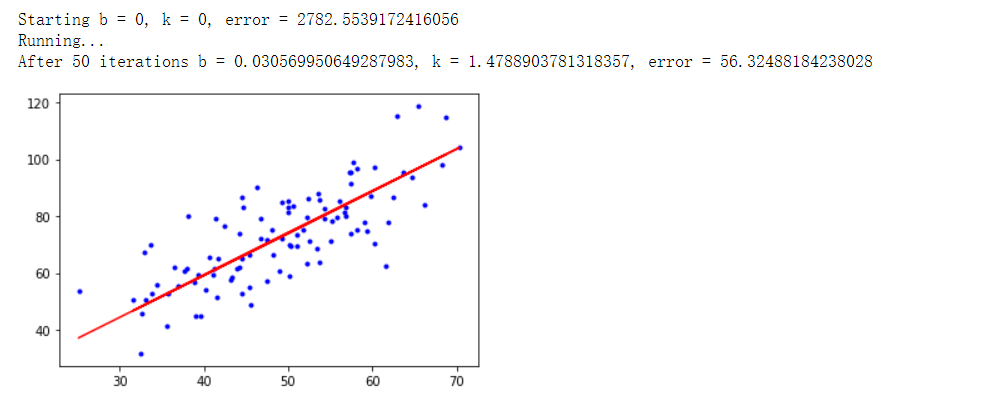梯度下降法-多元线性回归

import numpy as np
from numpy import genfromtxt
import matplotlib.pyplot as plt
from mpl_toolkits.mplot3d import Axes3D

# 读入数据
data = genfromtxt(r"Delivery.csv",delimiter=',')

# 切分数据
x_data = data[:,:-1]
y_data = data[:,-1]

# 学习率learning rate
lr = 0.0001
# 参数
theta0 = 0
theta1 = 0
theta2 = 0
# 最大迭代次数
epochs = 1000

# 最小二乘法
def compute_error(theta0, theta1, theta2, x_data, y_data):
totalError = 0
for i in range(0, len(x_data)):
totalError += (y_data[i] - (theta1 * x_data[i,0] + theta2*x_data[i,1] + theta0)) ** 2

def gradient_descent_runner(x_data, y_data, theta0, theta1, theta2, lr, epochs):
# 计算总数据量
m = float(len(x_data))
# 循环epochs次
for i in range(epochs):
# 计算梯度的总和再求平均
for j in range(0, len(x_data)):
theta0_grad += (1/m) * ((theta1 * x_data[j,0] + theta2*x_data[j,1] + theta0) - y_data[j])
theta1_grad += (1/m) * x_data[j,0] * ((theta1 * x_data[j,0] + theta2*x_data[j,1] + theta0) - y_data[j])
theta2_grad += (1/m) * x_data[j,1] * ((theta1 * x_data[j,0] + theta2*x_data[j,1] + theta0) - y_data[j])
# 更新b和k
theta0 = theta0 - (lr*theta0_grad)
theta1 = theta1 - (lr*theta1_grad)
theta2 = theta2 - (lr*theta2_grad)
return theta0, theta1, theta2

print("Starting theta0 = {0}, theta1 = {1}, theta2 = {2}, error = {3}".
format(theta0, theta1, theta2, compute_error(theta0, theta1, theta2, x_data, y_data)))
print("Running...")
theta0, theta1, theta2 = gradient_descent_runner(x_data, y_data, theta0, theta1, theta2, lr, epochs)
print("After {0} iterations theta0 = {1}, theta1 = {2}, theta2 = {3}, error = {4}".
format(epochs, theta0, theta1, theta2, compute_error(theta0, theta1, theta2, x_data, y_data)))

ax = plt.figure().add_subplot(111, projection = '3d')
ax.scatter(x_data[:,0], x_data[:,1], y_data, c = 'r', marker = 'o', s = 100) #点为红色三角形
x0 = x_data[:,0]
x1 = x_data[:,1]
# 生成网格矩阵
x0, x1 = np.meshgrid(x0, x1)
z = theta0 + x0*theta1 + x1*theta2
# 画3D图
ax.plot_surface(x0, x1, z)
#设置坐标轴
ax.set_xlabel('Miles')
ax.set_ylabel('Num of Deliveries')
ax.set_zlabel('Time')

#显示图像
plt.show()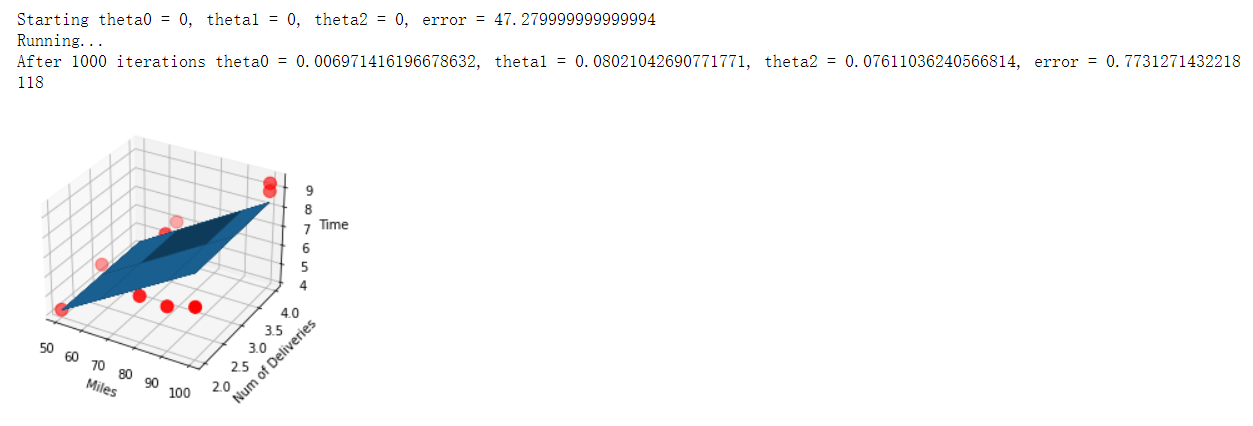## 2. 逻辑回归

逻辑回归原理与推导
梯度下降法-逻辑回归

import matplotlib.pyplot as plt
import numpy as np
from sklearn.metrics import classification_report
from sklearn import preprocessing
# 数据是否需要标准化
scale = True

# 载入数据
data = np.genfromtxt("LR-testSet.csv", delimiter=",")
x_data = data[:,:-1]
y_data = data[:,-1]

def plot():
x0 = []
x1 = []
y0 = []
y1 = []
# 切分不同类别的数据
for i in range(len(x_data)):
if y_data[i]==0:
x0.append(x_data[i,0])
y0.append(x_data[i,1])
else:
x1.append(x_data[i,0])
y1.append(x_data[i,1])

# 画图
scatter0 = plt.scatter(x0, y0, c='b', marker='o')
scatter1 = plt.scatter(x1, y1, c='r', marker='x')
#画图例
plt.legend(handles=[scatter0,scatter1],labels=['label0','label1'],loc='best')

plot()
#查看数据
plt.show()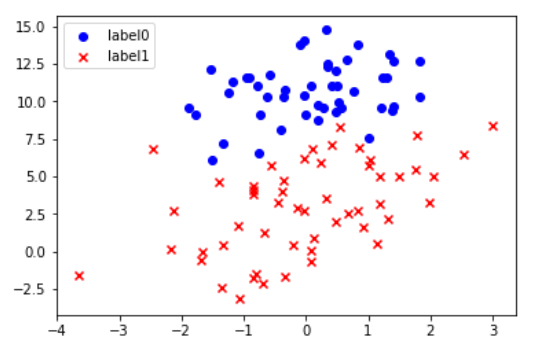# 数据处理，添加偏置项
x_data = data[:,:-1]
y_data = data[:,-1,np.newaxis]

print(np.mat(x_data).shape)
print(np.mat(y_data).shape)
# 给样本添加偏置项
X_data = np.concatenate((np.ones((100,1)),x_data),axis=1)

def sigmoid(x):
return 1.0/(1+np.exp(-x))

def cost(xMat, yMat, ws):
left = np.multiply(yMat, np.log(sigmoid(xMat*ws)))
right = np.multiply(1 - yMat, np.log(1 - sigmoid(xMat*ws)))
return np.sum(left + right) / -(len(xMat))

if scale == True:
xArr = preprocessing.scale(xArr)
xMat = np.mat(xArr)
yMat = np.mat(yArr)

lr = 0.001
epochs = 10000
costList = []
# 计算数据行列数
# 行代表数据个数，列代表权值个数
m,n = np.shape(xMat)
# 初始化权值
ws = np.mat(np.ones((n,1)))

for i in range(epochs+1):
# xMat和weights矩阵相乘
h = sigmoid(xMat*ws)
# 计算误差
ws_grad = xMat.T*(h - yMat)/m
ws = ws - lr*ws_grad

if i % 50 == 0:
costList.append(cost(xMat,yMat,ws))
return ws,costList

# 训练模型，得到权值和cost值的变化
ws,costList = gradAscent(X_data, y_data)
print(ws)

if scale == False:
# 画图决策边界
plot()
x_test = [[-4],]
y_test = (-ws - x_test*ws)/ws
plt.plot(x_test, y_test, 'k')
plt.show()

# 画图 loss值的变化
x = np.linspace(0,10000,201)
plt.plot(x, costList, c='r')
plt.title('Train')
plt.xlabel('Epochs')
plt.ylabel('Cost')
plt.show()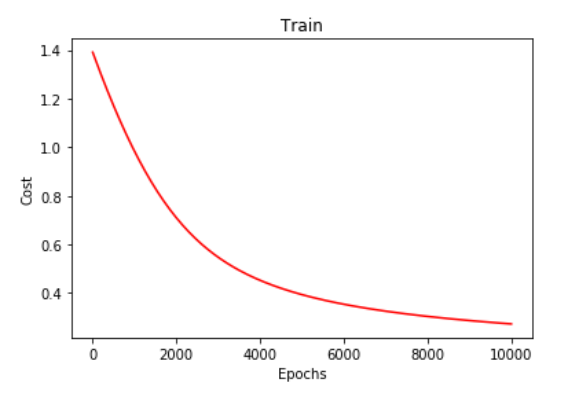# 预测
def predict(x_data, ws):
if scale == True:
x_data = preprocessing.scale(x_data)
xMat = np.mat(x_data)
ws = np.mat(ws)
return [1 if x >= 0.5 else 0 for x in sigmoid(xMat*ws)]

predictions = predict(X_data, ws)

print(classification_report(y_data, predictions))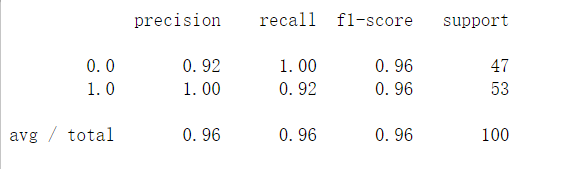梯度下降法-非线性逻辑回归

import matplotlib.pyplot as plt
import numpy as np
from sklearn.metrics import classification_report
from sklearn import preprocessing
from sklearn.preprocessing import PolynomialFeatures
# 数据是否需要标准化
scale = False

# 载入数据
data = np.genfromtxt("LR-testSet2.txt", delimiter=",")
x_data = data[:,:-1]
y_data = data[:,-1,np.newaxis]

def plot():
x0 = []
x1 = []
y0 = []
y1 = []
# 切分不同类别的数据
for i in range(len(x_data)):
if y_data[i]==0:
x0.append(x_data[i,0])
y0.append(x_data[i,1])
else:
x1.append(x_data[i,0])
y1.append(x_data[i,1])

# 画图
scatter0 = plt.scatter(x0, y0, c='b', marker='o')
scatter1 = plt.scatter(x1, y1, c='r', marker='x')
#画图例
plt.legend(handles=[scatter0,scatter1],labels=['label0','label1'],loc='best')

plot()
plt.show()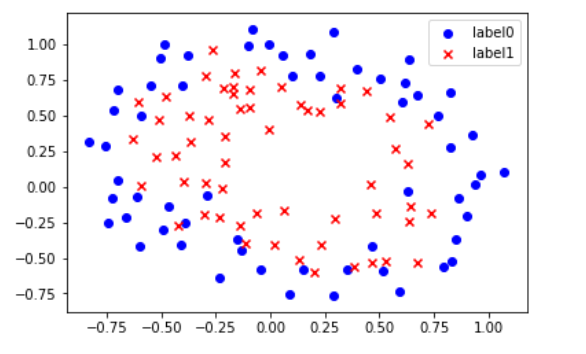# 定义多项式回归,degree的值可以调节多项式的特征
poly_reg  = PolynomialFeatures(degree=3)
# 特征处理
x_poly = poly_reg.fit_transform(x_data)

def sigmoid(x):
return 1.0/(1+np.exp(-x))

def cost(xMat, yMat, ws):
left = np.multiply(yMat, np.log(sigmoid(xMat*ws)))
right = np.multiply(1 - yMat, np.log(1 - sigmoid(xMat*ws)))
return np.sum(left + right) / -(len(xMat))

if scale == True:
xArr = preprocessing.scale(xArr)
xMat = np.mat(xArr)
yMat = np.mat(yArr)

lr = 0.03
epochs = 50000
costList = []
# 计算数据列数，有几列就有几个权值
m,n = np.shape(xMat)
# 初始化权值
ws = np.mat(np.ones((n,1)))

for i in range(epochs+1):
# xMat和weights矩阵相乘
h = sigmoid(xMat*ws)
# 计算误差
ws_grad = xMat.T*(h - yMat)/m
ws = ws - lr*ws_grad

if i % 50 == 0:
costList.append(cost(xMat,yMat,ws))
return ws,costList

# 训练模型，得到权值和cost值的变化
ws,costList = gradAscent(x_poly, y_data)
print(ws)

# 获取数据值所在的范围
x_min, x_max = x_data[:, 0].min() - 1, x_data[:, 0].max() + 1
y_min, y_max = x_data[:, 1].min() - 1, x_data[:, 1].max() + 1

# 生成网格矩阵
xx, yy = np.meshgrid(np.arange(x_min, x_max, 0.02),
np.arange(y_min, y_max, 0.02))

# np.r_按row来组合array，
# np.c_按colunm来组合array
# >>> a = np.array([1,2,3])
# >>> b = np.array([5,2,5])
# >>> np.r_[a,b]
# array([1, 2, 3, 5, 2, 5])
# >>> np.c_[a,b]
# array([[1, 5],
#        [2, 2],
#        [3, 5]])
# >>> np.c_[a,[0,0,0],b]
# array([[1, 0, 5],
#        [2, 0, 2],
#        [3, 0, 5]])
z = sigmoid(poly_reg.fit_transform(np.c_[xx.ravel(), yy.ravel()]).dot(np.array(ws)))# ravel与flatten类似，多维数据转一维。flatten不会改变原始数据，ravel会改变原始数据
for i in range(len(z)):
if z[i] > 0.5:
z[i] = 1
else:
z[i] = 0
z = z.reshape(xx.shape)

# 等高线图
cs = plt.contourf(xx, yy, z)
plot()
plt.show()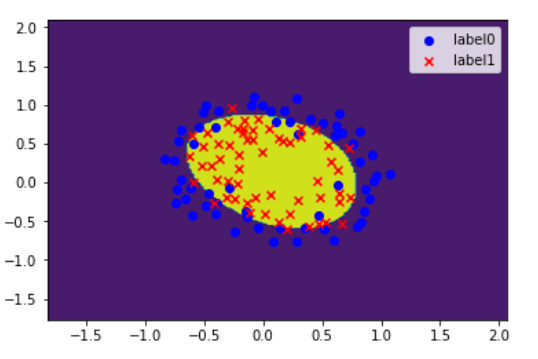# 预测
def predict(x_data, ws):
#     if scale == True:
#         x_data = preprocessing.scale(x_data)
xMat = np.mat(x_data)
ws = np.mat(ws)
return [1 if x >= 0.5 else 0 for x in sigmoid(xMat*ws)]

predictions = predict(x_poly, ws)

print(classification_report(y_data, predictions))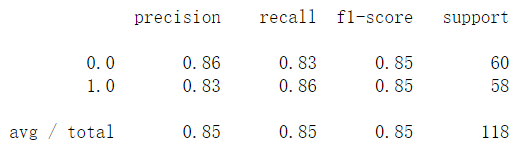神经网络

## 4. SVM支持向量机

SVM-非线性

import matplotlib.pyplot as plt
import numpy as np
from sklearn.metrics import classification_report
from sklearn import svm

# 载入数据
data = np.genfromtxt("LR-testSet2.txt", delimiter=",")
x_data = data[:,:-1]
y_data = data[:,-1]

def plot():
x0 = []
x1 = []
y0 = []
y1 = []
# 切分不同类别的数据
for i in range(len(x_data)):
if y_data[i]==0:
x0.append(x_data[i,0])
y0.append(x_data[i,1])
else:
x1.append(x_data[i,0])
y1.append(x_data[i,1])

# 画图
scatter0 = plt.scatter(x0, y0, c='b', marker='o')
scatter1 = plt.scatter(x1, y1, c='r', marker='x')
#画图例
plt.legend(handles=[scatter0,scatter1],labels=['label0','label1'],loc='best')

plot()
plt.show()

# fit the model
# C和gamma
model = svm.SVC(kernel='rbf')
model.fit(x_data, y_data)

model.score(x_data,y_data)

# 获取数据值所在的范围
x_min, x_max = x_data[:, 0].min() - 1, x_data[:, 0].max() + 1
y_min, y_max = x_data[:, 1].min() - 1, x_data[:, 1].max() + 1

# 生成网格矩阵
xx, yy = np.meshgrid(np.arange(x_min, x_max, 0.02),
np.arange(y_min, y_max, 0.02))

z = model.predict(np.c_[xx.ravel(), yy.ravel()])# ravel与flatten类似，多维数据转一维。flatten不会改变原始数据，ravel会改变原始数据
z = z.reshape(xx.shape)

# 等高线图
cs = plt.contourf(xx, yy, z)
plot()
plt.show()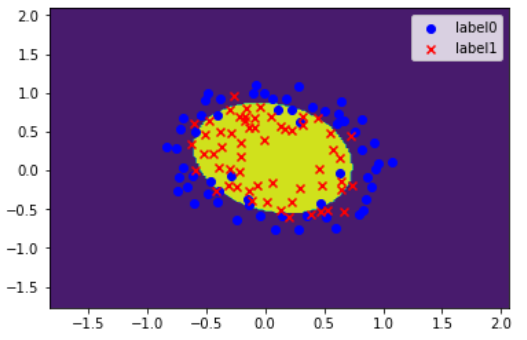## 5. K邻近

主要过程案例

# 导入算法包以及数据集
import numpy as np
from sklearn import datasets
from sklearn.model_selection import train_test_split
from tqdm.notebook import tqdm
from sklearn.metrics import classification_report,confusion_matrix
import operator
import random

def knn(x_test, x_data, y_data, k):
# 计算样本数量
x_data_size = x_data.shape
# 复制x_test
np.tile(x_test, (x_data_size,1))
# 计算x_test与每一个样本的差值
diffMat = np.tile(x_test, (x_data_size,1)) - x_data
# 计算差值的平方
sqDiffMat = diffMat**2
# 求和
sqDistances = sqDiffMat.sum(axis=1)
# 开方
distances = sqDistances**0.5
# 从小到大排序
sortedDistances = distances.argsort()
classCount = {}
for i in range(k):
# 获取标签
votelabel = y_data[sortedDistances[i]]
# 统计标签数量
classCount[votelabel] = classCount.get(votelabel,0) + 1
# 根据operator.itemgetter(1)-第1个值对classCount排序，然后再取倒序
sortedClassCount = sorted(classCount.items(),key=operator.itemgetter(1), reverse=True)
# 获取数量最多的标签
return sortedClassCount

# 载入数据

#打乱数据
data_size = iris.data.shape
index = [i for i in range(data_size)]
random.shuffle(index)
iris.data = iris.data[index]
iris.target = iris.target[index]

#切分数据集
test_size = 40
x_train = iris.data[test_size:]
x_test =  iris.data[:test_size]
y_train = iris.target[test_size:]
y_test = iris.target[:test_size]

#分类
predictions = []
for i in tqdm(range(x_test.shape)):
predictions.append(knn(x_test[i], x_train, y_train, 5))

#评估
target_names = ['class 0', 'class 1', 'class 2']
print(classification_report(y_test, predictions,target_names=target_names))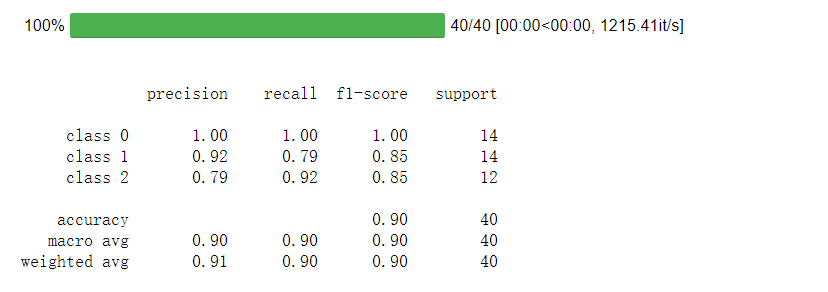## 6. 贝叶斯

设每个数据样本用一个n维特征向量来描述n个属性的值，即：X={x1，x2，…，xn}，假定有m个类，分别用C1, C2,…，Cm表示。给定一个未知的数据样本X（即没有类标号），若朴素贝叶斯分类法将未知的样本X分配给类Ci，则一定是
P(Ci|X)>P(Cj|X) 1≤j≤m，j≠i

from sklearn.naive_bayes import  GaussianNB
from sklearn.model_selection import train_test_split
from sklearn.metrics import accuracy_score
from sklearn.preprocessing import LabelEncoder
import pandas as pd
from numpy import *
import operator
#计算高斯分布密度函数的值
def calculate_gaussian_probability(mean, var, x):
coeff = (1.0 / (math.sqrt((2.0 * math.pi) * var)))
exponent = math.exp(-(math.pow(x - mean, 2) / (2 * var)))
c= coeff * exponent
return c
#计算均值
def averagenum(num):
nsum = 0
for i in range(len(num)):
nsum += num[i]
return nsum / len(num)
#计算方差
def var(list,avg):
var1=0
for i in list:
var1+=float((i-avg)**2)
var2=(math.sqrt(var1/(len(list)*1.0)))
return var2
#朴素贝叶斯分类模型
def Naivebeys(splitData, classset, test):
classify = []
for s in range(len(test)):
c = {}
for i in classset:
splitdata = splitData[i]
num = len(splitdata)
mu = num + 2
character = len(splitdata)-1    #具体数据集，个数有变
classp = []
for j in range(character):
zi = 1
if isinstance(splitdata[j], (int, float)):
numlist=[example[j] for example in splitdata]
Mean=averagenum(numlist)
Var=var(numlist,Mean)
a = calculate_gaussian_probability(Mean, Var, test[s][j])
else:
for l in range(num):
if test[s][j] == splitdata[l][j]:
zi += 1
a=zi/mu
classp.append(a)
zhi = 1
for k in range(character):
zhi *= classp[k]
c.setdefault(i, zhi)
sorta = sorted(c.items(), key=operator.itemgetter(1), reverse=True)
classify.append(sorta)
return classify
#评估
def accuracy(y, y_pred):
yarr=array(y)
y_predarr=array(y_pred)
yarr = yarr.reshape(yarr.shape, -1)
y_predarr = y_predarr.reshape(y_predarr.shape, -1)
return sum(yarr == y_predarr) / len(yarr)
#数据处理
def splitDataset(dataSet):   #按照属性把数据划分
classList = [example[-1] for example in dataSet]
classSet = set(classList)
splitDir = {}
for i in classSet:
for j in range(len(dataSet)):
if dataSet[j][-1] == i:
splitDir.setdefault(i, []).append(dataSet[j])
return splitDir, classSet

open('test.txt')
class_le = LabelEncoder()
dataSet = df.values[:, :]
dataset_train,dataset_test=train_test_split(dataSet, test_size=0.1)
splitDataset_train, classSet_train = splitDataset(dataset_train)
classSet_test=[example[-1] for example in dataset_test]
y_pred= Naivebeys(splitDataset_train, classSet_train, dataset_test)
accu=accuracy(classSet_test,y_pred)
print("Accuracy:", accu)


Accuracy: 0.65

## 7. 决策树

决策树的分类模型是树状结构，简单直观，比较符合人类的理解方式。决策树分类器的构造不需要任何领域知识和参数设置，适合于探索式知识的发现。由于决策树分类步骤简单快速，而且一般来说具有较高的准确率，因此得到了较多的使用。

信息量
某事件发生所含有的信息量是该事件发生概率的函数：其中， p ( x i ) p(x_{i}) x i x_{i} 发生的概率， I ( x i ) I(x_{i}) 表示 x i x_{i} 发生所含的信息量，称为 x i x_{i} 的自信息量，单位是比特 ( b ) (b)
I ( x i ) = − log ⁡ 2 p ( x i ) I(x_{i})=-\log_2 p(x_{i})
信息熵
如果将信息源所有可能事件的自信息量进行平均，即可得到信息的“熵”。设信息源 X X 的符号集为 x i ( i = 1 , 2 , ⋯   , N ) x_{i}(i=1,2,\cdots,N) x i x_{i} 出现的概率为 p ( x i ) p(x_{i}) ，则信息源 X X 的熵为：
H ( X ) = ∑ i = 1 N p ( x i ) I ( x i ) = − ∑ i = 1 N p ( x i ) log ⁡ 2 p ( x i ) H(X)=\sum_{i=1}^{N}p(x_{i})I(x_{i})=-\sum_{i=1}^{N}p(x_{i})\log_2 p(x_{i})
ID3算法
ID3算法是Quinlan于1986年提出的，只能处理离散型描述属性，在选择根节点和各个内部节点上的分枝属性时，采用信息增益作为度量标准，选择具有最高信息增益的描述属性作为分枝属性。

假设 n j n_{j} 是数据集 X X 中属于类别 c j c_{j} 的样本数量，则各类别的先验概率为 p ( c j ) = n j t o t a l ， j = 1 , 2 , ⋯   , m p(c_{j})=\frac{n_{j}}{total}，j=1,2,\cdots,m

数据集 X X 的期望信息为：
I ( n 1 , n 2 , ⋯   , n m ) = − ∑ i = 1 N p ( c j ) log ⁡ 2 p ( c j ) I(n_{1},n_{2},\cdots,n_{m})=-\sum_{i=1}^{N}p(c_{j})\log_2 p(c_{j})

由描述属性 A f A_{f} 划分数据集 X X 所得的熵为：
E ( A f ) = ∑ s = 1 q n 1 s + ⋯ + n m s t o t a l I ( n 1 s , ⋯   , n m s ) E(A_{f})=\sum_{s=1}^{q}\frac{n_{1s}+\cdots+n_{ms}}{total}I(n_{1s},\cdots,n_{ms})

其中：
I ( n 1 s , ⋯   , n m s ) = − ∑ j = 1 m p j s log ⁡ 2 p j s I(n_{1s},\cdots,n_{ms})=-\sum_{j=1}^{m}p_{js}\log_2 p_{js}
p j s = n j s n s p_{js}=\frac{n_{js}}{n_{s}}

Af划分数据集产生的信息增益为：
G a i n ( A f ) = I ( n 1 , n 2 , ⋯   , n m ) − E ( A f ) Gain(A_{f})=I(n_{1},n_{2},\cdots,n_{m})-E(A_{f})

数据集介绍
本实验采用西瓜数据集，根据西瓜的几种属性判断西瓜是否是好瓜。数据集包含17条记录，数据格式如下：

色泽根蒂敲声纹理脐部触感好瓜
青绿蜷缩浊响清晰凹陷硬滑
乌黑蜷缩沉闷清晰凹陷硬滑
乌黑蜷缩浊响清晰凹陷硬滑
⋯ \cdots ⋯ \cdots ⋯ \cdots ⋯ \cdots ⋯ \cdots ⋯ \cdots ⋯ \cdots

实验
首先我们引入必要的库：

import pandas as pd
from math import log2
from pylab import *
import matplotlib.pyplot as plt


导入数据
读取csv文件中的数据记录并转为列表

def load_dataset():
# 数据集文件所在位置
path = "./西瓜.csv"
dataset = []
for a in data.values:
dataset.append(list(a))
# 返回数据列表
attribute = list(data.keys())
# 返回数据集和每个维度的名称
return dataset, attribute
attribute,dataset

(['色泽', '根蒂', '敲声', '纹理', '脐部', '触感', '好瓜'],
[['青绿', '蜷缩', '浊响', '清晰', '凹陷', '硬滑', '是'],
['乌黑', '蜷缩', '沉闷', '清晰', '凹陷', '硬滑', '是'],
['乌黑', '蜷缩', '浊响', '清晰', '凹陷', '硬滑', '是'],
['青绿', '蜷缩', '沉闷', '清晰', '凹陷', '硬滑', '是'],
['浅白', '蜷缩', '浊响', '清晰', '凹陷', '硬滑', '是'],
['青绿', '稍蜷', '浊响', '清晰', '稍凹', '软粘', '是'],
['乌黑', '稍蜷', '浊响', '稍糊', '稍凹', '软粘', '是'],
['乌黑', '稍蜷', '浊响', '清晰', '稍凹', '硬滑', '是'],
['乌黑', '稍蜷', '沉闷', '稍糊', '稍凹', '硬滑', '否'],
['青绿', '硬挺', '清脆', '清晰', '平坦', '软粘', '否'],
['浅白', '硬挺', '清脆', '模糊', '平坦', '硬滑', '否'],
['浅白', '蜷缩', '浊响', '模糊', '平坦', '软粘', '否'],
['青绿', '稍蜷', '浊响', '稍糊', '凹陷', '硬滑', '否'],
['浅白', '稍蜷', '沉闷', '稍糊', '凹陷', '硬滑', '否'],
['乌黑', '稍蜷', '浊响', '清晰', '稍凹', '软粘', '否'],
['浅白', '蜷缩', '浊响', '模糊', '平坦', '硬滑', '否'],
['青绿', '蜷缩', '沉闷', '稍糊', '稍凹', '硬滑', '否']])


计算信息熵

def calculate_info_entropy(dataset):
# 记录样本数量
n = len(dataset)
# 记录分类属性数量
attribute_count = {}
# 遍历所有实例，统计类别出现频次
for attribute in dataset:
# 每一个实例最后一列为类别属性，因此取最后一列
class_attribute = attribute[-1]
# 如果当前类标号不在label_count中，则加入该类标号
if class_attribute not in attribute_count.keys():
attribute_count[class_attribute] = 0
# 类标号出现次数加1
attribute_count[class_attribute] += 1
info_entropy = 0
for class_attribute in attribute_count:
# 计算该类在实例中出现的概率
p = float(attribute_count[class_attribute]) / n
info_entropy -= p * log2(p)
return info_entropy


数据集划分

def split_dataset(dataset,i,value):
split_set = []
for attribute in dataset:
if attribute[i] == value:
# 删除该维属性
reduce_attribute = attribute[:i]
reduce_attribute.extend(attribute[i+1:])
split_set.append(reduce_attribute)
return split_set


计算属性划分数据集的熵

def calculate_attribute_entropy(dataset,i,values):
attribute_entropy = 0
for value in values:
sub_dataset = split_dataset(dataset,i,value)
p = len(sub_dataset) / float(len(dataset))
attribute_entropy += p*calculate_info_entropy(sub_dataset)
return attribute_entropy


计算信息增益

def calculate_info_gain(dataset,info_entropy,i):
# 第i维特征列表
attribute = [example[i] for example in dataset]
# 转为不重复元素的集合
values = set(attribute)
attribute_entropy = calculate_attribute_entropy(dataset,i,values)
info_gain = info_entropy - attribute_entropy
return info_gain


根据信息增益进行划分

def split_by_info_gain(dataset):
# 描述属性数量
attribute_num = len(dataset) - 1
# 整个数据集的信息熵
info_entropy = calculate_info_entropy(dataset)
# 最高的信息增益
max_info_gain = 0
# 最佳划分维度属性
best_attribute = -1
for i in range(attribute_num):
info_gain = calculate_info_gain(dataset,info_entropy,i)
if(info_gain > max_info_gain):
max_info_gain = info_gain
best_attribute = i
return best_attribute


构造决策树

def create_tree(dataset,attribute):
# 类别列表
class_list = [example[-1] for example in dataset]
# 统计类别class_list的数量
if class_list.count(class_list) == len(class_list):
# 当类别相同则停止划分
return class_list
# 最佳划分维度对应的索引
best_attribute = split_by_info_gain(dataset)
# 最佳划分维度对应的名称
best_attribute_name = attribute[best_attribute]
tree = {best_attribute_name:{}}
del(attribute[best_attribute])
# 查找需要分类的特征子集
attribute_values = [example[best_attribute] for example in dataset]
values = set(attribute_values)
for value in values:
sub_attribute = attribute[:]
tree[best_attribute_name][value] =create_tree(split_dataset(dataset,best_attribute,value),sub_attribute)
return tree
tree = create_tree(dataset,attribute)
tree

{'纹理': {'清晰': {'根蒂': {'蜷缩': '是',
'硬挺': '否',
'稍蜷': {'色泽': {'青绿': '是', '乌黑': {'触感': {'软粘': '否', '硬滑': '是'}}}}}},
'模糊': '否',
'稍糊': {'触感': {'软粘': '是', '硬滑': '否'}}}}

# 定义划分属性节点样式
attribute_node = dict(boxstyle="round", color='#00B0F0')
# 定义分类属性节点样式
class_node = dict(boxstyle="circle", color='#00F064')
# 定义箭头样式
arrow = dict(arrowstyle="<-", color='#000000')

# 计算叶结点数
def get_num_leaf(tree):
numLeafs = 0
firstStr = list(tree.keys())
secondDict = tree[firstStr]
for key in secondDict.keys():
if type(secondDict[key]).__name__ == 'dict':
numLeafs += get_num_leaf(secondDict[key])
else:
numLeafs += 1
return numLeafs

# 计算树的层数
def get_depth_tree(tree):
maxDepth = 0
firstStr = list(tree.keys())
secondDict = tree[firstStr]
for key in secondDict.keys():
if type(secondDict[key]).__name__ == 'dict':
thisDepth = 1 + get_depth_tree(secondDict[key])
else:
thisDepth = 1
if thisDepth > maxDepth:
maxDepth = thisDepth
return maxDepth

# 绘制文本框
def plot_text(cntrPt, parentPt, txtString):
xMid = (parentPt - cntrPt) / 2.0 + cntrPt
yMid = (parentPt - cntrPt) / 2.0 + cntrPt
createPlot.ax1.text(xMid, yMid, txtString, va="center", ha="center", rotation=30)


绘制树结构

def plotTree(tree, parentPt, nodeTxt):
numLeafs = get_num_leaf(tree)
depth = get_depth_tree(tree)
firstStr = list(tree.keys())
cntrPt = (plotTree.xOff + (1.0 + float(numLeafs)) / 2.0 / plotTree.totalW, plotTree.yOff)
plot_text(cntrPt, parentPt, nodeTxt)  #在父子结点间绘制文本框并填充文本信息
plotNode(firstStr, cntrPt, parentPt, attribute_node)  #绘制带箭头的注释
secondDict = tree[firstStr]
plotTree.yOff = plotTree.yOff - 1.0 / plotTree.totalD
for key in secondDict.keys():
if type(secondDict[key]).__name__ == 'dict':
plotTree(secondDict[key], cntrPt, str(key))
else:
plotTree.xOff = plotTree.xOff + 1.0 / plotTree.totalW
plotNode(secondDict[key], (plotTree.xOff, plotTree.yOff), cntrPt, class_node)
plot_text((plotTree.xOff, plotTree.yOff), cntrPt, str(key))
plotTree.yOff = plotTree.yOff + 1.0 / plotTree.totalD

# 绘制箭头
def plotNode(nodeTxt, centerPt, parentPt, nodeType):
createPlot.ax1.annotate(nodeTxt, xy=parentPt, xycoords='axes fraction',
xytext=centerPt, textcoords='axes fraction',
va="center", ha="center", bbox=nodeType, arrowprops=arrow)

# 绘图
def createPlot(tree):
fig = plt.figure(1, facecolor='white')
fig.clf()
axprops = dict(xticks=[], yticks=[])
createPlot.ax1 = plt.subplot(111, frameon=False, **axprops)
plotTree.totalW = float(get_num_leaf(tree))
plotTree.totalD = float(get_depth_tree(tree))
plotTree.xOff = -0.5 / plotTree.totalW;
plotTree.yOff = 1.0;
plotTree(tree, (0.5, 1.0), '')
plt.show()

#指定默认字体
mpl.rcParams['font.sans-serif'] = ['SimHei']
mpl.rcParams['axes.unicode_minus'] = False
# 绘制决策树
createPlot(tree)集成学习（Ensemble Learning），就是使用一系列学习器进行学习，并使用某种规则将各个学习器的结果进行整合，从而获得比单个学习器效果更好的学习效果的一种方法。

集成学习的条件
通过集成学习提高分类器的整体泛化能力有以下两个条件：

1. 基分类器之间具有差异性。如果使用的是同一个分类器集成，集成分类器的性能是不会有提升的。
2. 每个基分类器的分类精度必须大于0.5。如下图所示，当基分类器精度小于0.5时，随着集成规模的增加，分类集成分类器的分类精度会下降；但是如果基分类器的精度大于0.5时，集成分类器的最终分类精度是趋近于1的。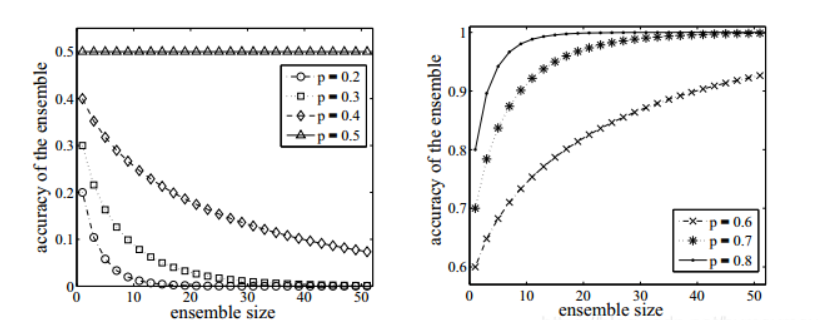集成学习的两个关键点：

1. 如何构建具有差异性的基分类器
2. 如何对基分类器的结果进行整合

构建差异性分类器一般有以下三种方法：

1. 通过处理数据集生成差异性分类器
2. 通过处理数据特征构建差异性分类器
3. 对分类器的处理构建差异性分类器

集成学习常见的三种元算法是Bagging, Boosting和Stacking。Bagging用于提升机器学习算法的稳定性和准确性。Boosting主要用于减少bias（偏差）和variance（方差），是将一个弱分类器转化为强分类器的算法。Stacking是一种组合多个模型的方法。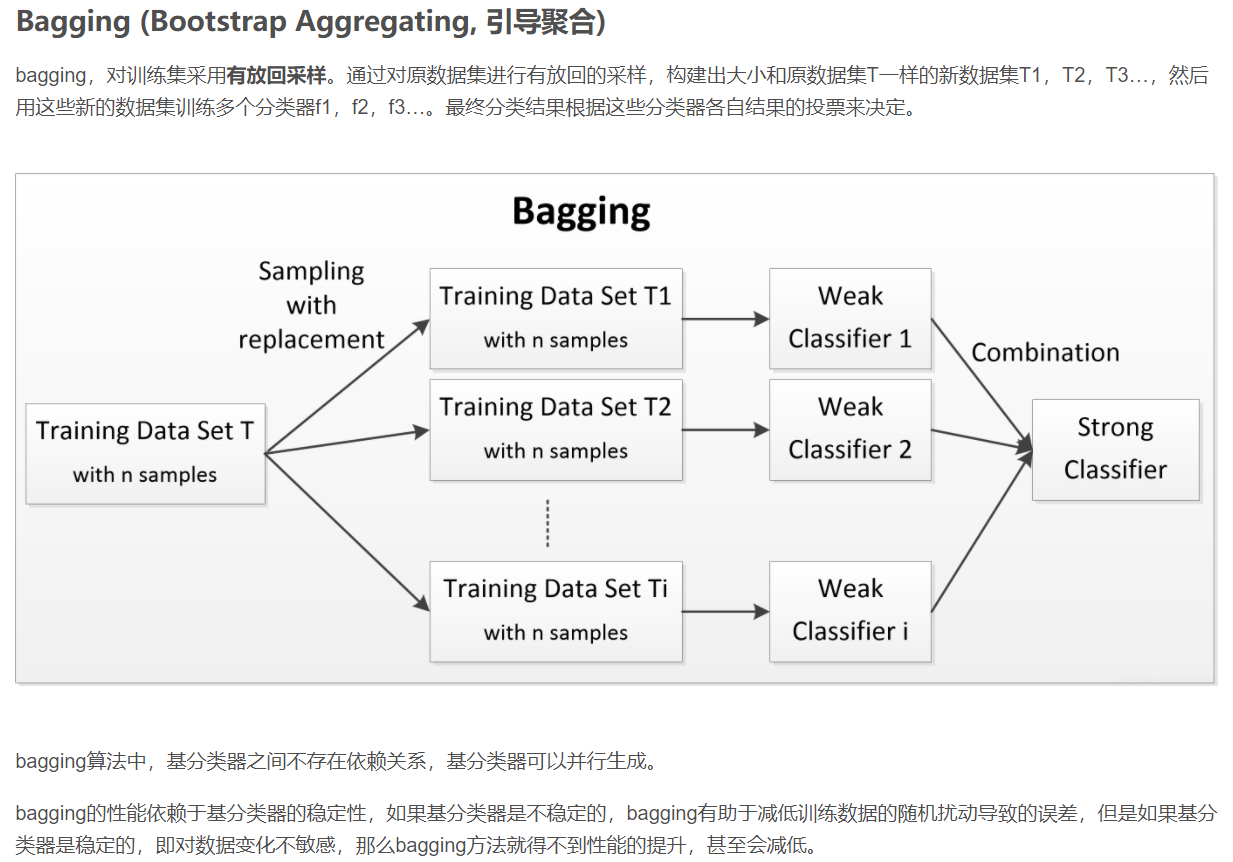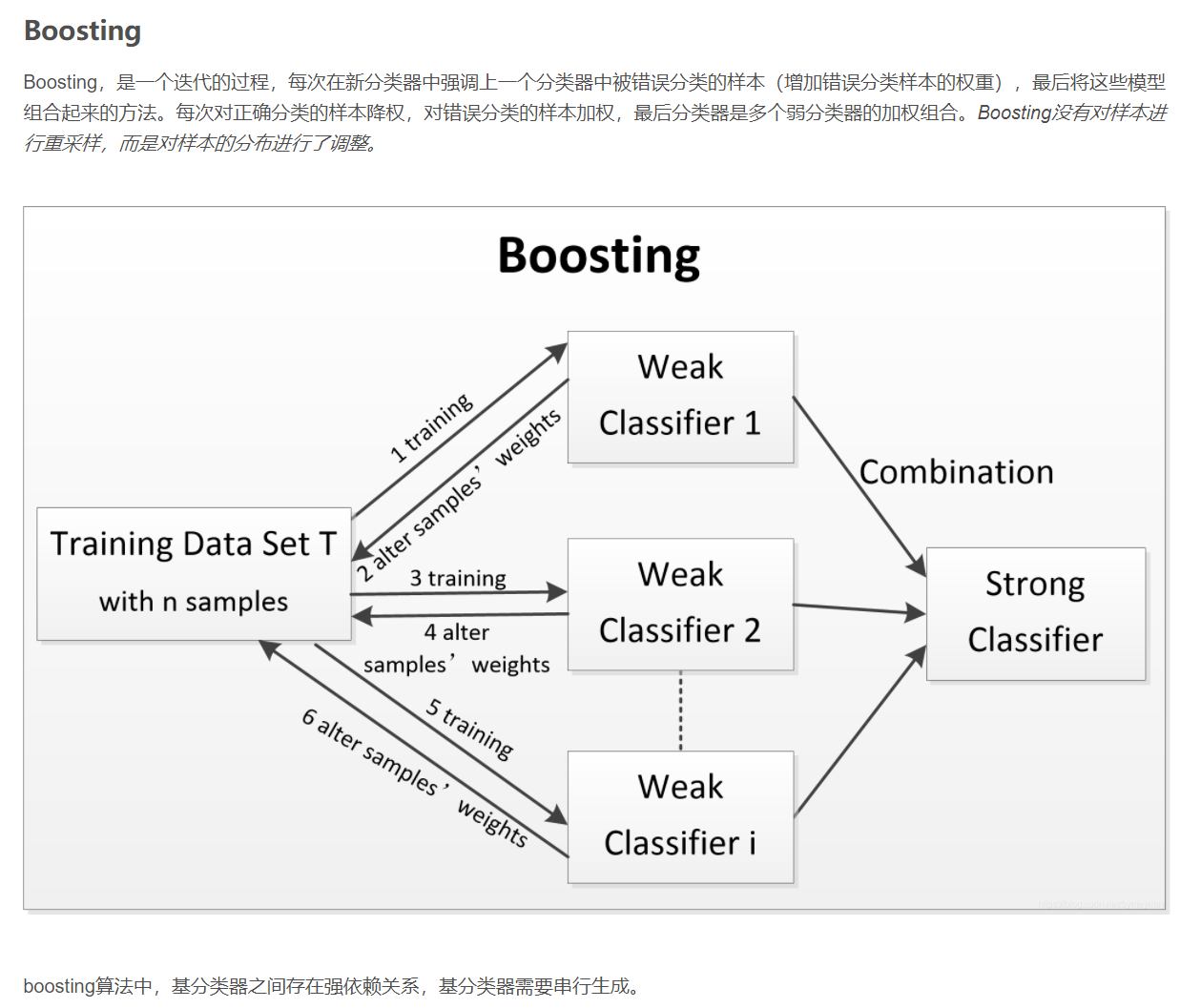Boosting分类方法，其过程如下所示：

1）先通过对N个训练数据的学习得到第一个弱分类器h1；

2）将h1分错的数据和其他的新数据一起构成一个新的有N个训练数据的样本，通过对这个样本的学习得到第二个弱分类器h2；

3）将h1和h2都分错了的数据加上其他的新数据构成另一个新的有N个训练数据的样本，通过对这个样本的学习得到第三个弱分类器h3；

4）最终经过提升的强分类器h_final=Majority Vote(h1,h2,h3)。即某个数据被分为哪一类要通过h1,h2,h3的多数表决。

上述Boosting算法，存在两个问题：

①如何调整训练集，使得在训练集上训练弱分类器得以进行。

②如何将训练得到的各个弱分类器联合起来形成强分类器。

①使用加权后选取的训练数据代替随机选取的训练数据，这样将训练的焦点集中在比较难分的训练数据上。

②将弱分类器联合起来时，使用加权的投票机制代替平均投票机制。让分类效果好的弱分类器具有较大的权重，而分类效果差的分类器具有较小的权重。

import numpy as np
import matplotlib.pyplot as plt
from sklearn import tree
from sklearn.ensemble import AdaBoostClassifier
from sklearn.tree import DecisionTreeClassifier
from sklearn.datasets import make_gaussian_quantiles
from sklearn.metrics import classification_report

# 生成2维正态分布，生成的数据按分位数分为两类，500个样本,2个样本特征
x1, y1 = make_gaussian_quantiles(n_samples=500, n_features=2,n_classes=2)
# 生成2维正态分布，生成的数据按分位数分为两类，400个样本,2个样本特征均值都为3
x2, y2 = make_gaussian_quantiles(mean=(3, 3), n_samples=500, n_features=2, n_classes=2)
# 将两组数据合成一组数据
x_data = np.concatenate((x1, x2))
y_data = np.concatenate((y1, - y2 + 1))

plt.scatter(x_data[:, 0], x_data[:, 1], c=y_data)
plt.show()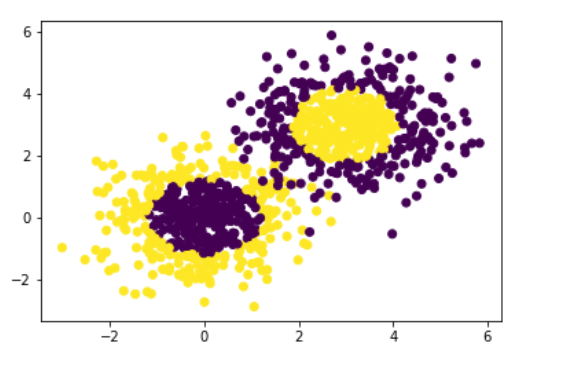# 决策树模型
model = tree.DecisionTreeClassifier(max_depth=3)

# 输入数据建立模型
model.fit(x_data, y_data)

# 获取数据值所在的范围
x_min, x_max = x_data[:, 0].min() - 1, x_data[:, 0].max() + 1
y_min, y_max = x_data[:, 1].min() - 1, x_data[:, 1].max() + 1

# 生成网格矩阵
xx, yy = np.meshgrid(np.arange(x_min, x_max, 0.02),
np.arange(y_min, y_max, 0.02))

z = model.predict(np.c_[xx.ravel(), yy.ravel()])# ravel与flatten类似，多维数据转一维。flatten不会改变原始数据，ravel会改变原始数据
z = z.reshape(xx.shape)
# 等高线图
cs = plt.contourf(xx, yy, z)
# 样本散点图
plt.scatter(x_data[:, 0], x_data[:, 1], c=y_data)
plt.show()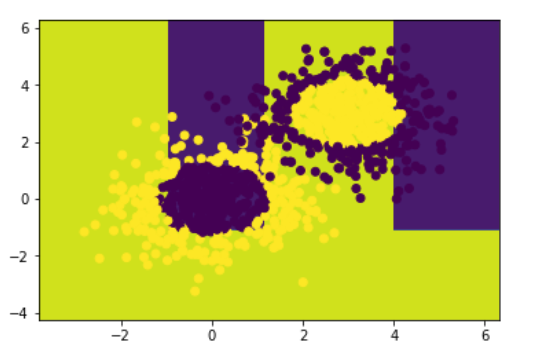# 模型准确率
model.score(x_data,y_data)

0.777

# AdaBoost模型
# 训练模型
model.fit(x_data, y_data)

# 获取数据值所在的范围
x_min, x_max = x_data[:, 0].min() - 1, x_data[:, 0].max() + 1
y_min, y_max = x_data[:, 1].min() - 1, x_data[:, 1].max() + 1

# 生成网格矩阵
xx, yy = np.meshgrid(np.arange(x_min, x_max, 0.02),
np.arange(y_min, y_max, 0.02))

# 获取预测值
z = model.predict(np.c_[xx.ravel(), yy.ravel()])
z = z.reshape(xx.shape)
# 等高线图
cs = plt.contourf(xx, yy, z)
# 样本散点图
plt.scatter(x_data[:, 0], x_data[:, 1], c=y_data)
plt.show()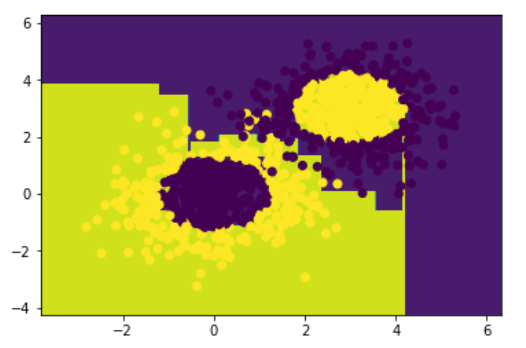# 模型准确率
model.score(x_data,y_data)

0.976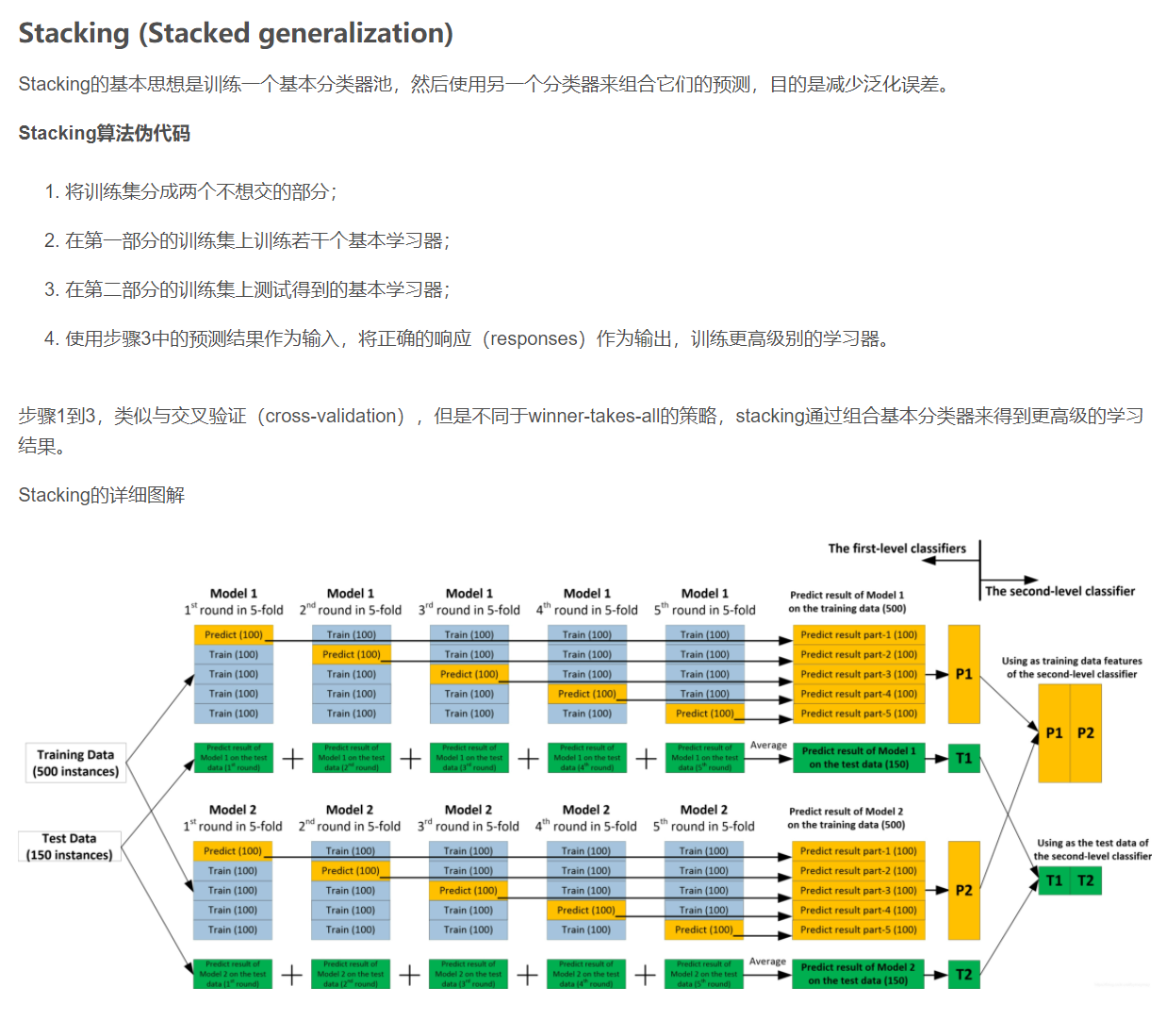总结一下，组合算法（combiner algorithm）使用所有其他算法的预测作为附加输入（additional inputs）来训练得到最终的预测结果。理论上可以表示任何一种组合学习方法（ensemble techniques）；实际中，单层的逻辑回归模型（single-layer logistic regression model）通常被用作组合器（combiner）。

# 非监督学习

## 9. 降维—主成分分析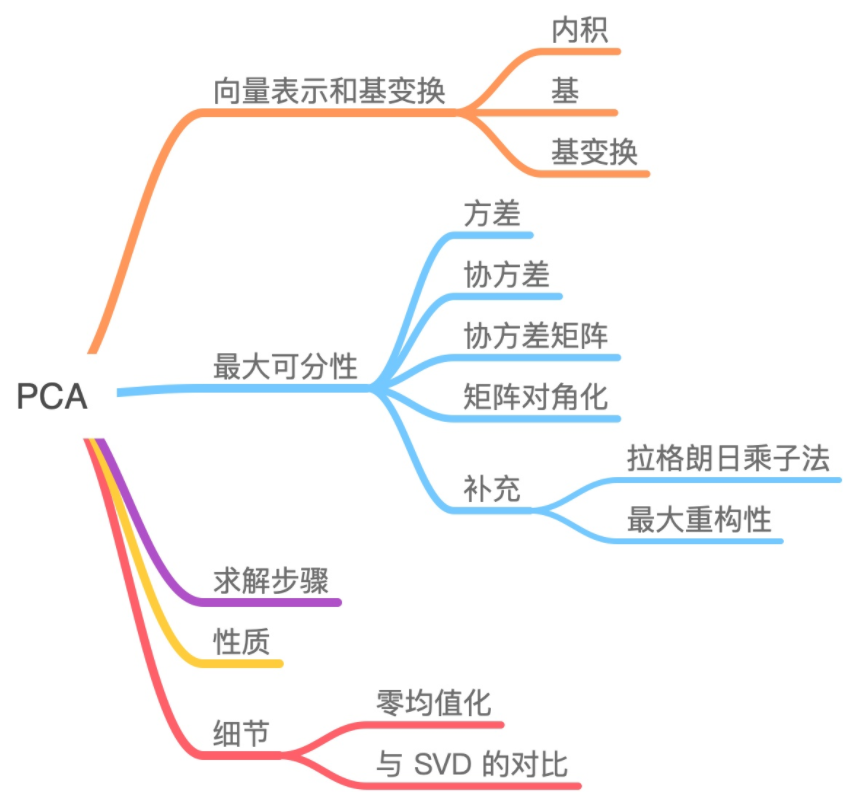import numpy as np
import matplotlib.pyplot as plt

# 载入数据
data = np.genfromtxt("data.csv", delimiter=",")
x_data = data[:,0]
y_data = data[:,1]
plt.scatter(x_data,y_data)
plt.show()
print(x_data.shape)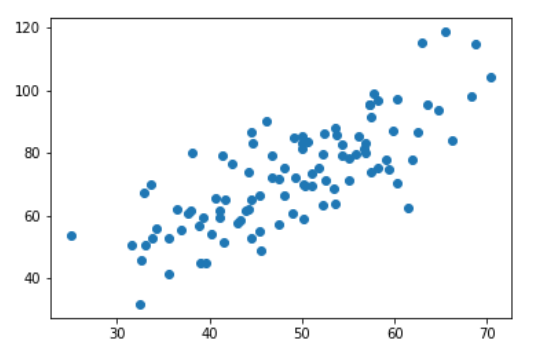# 数据中心化
def zeroMean(dataMat):
# 按列求平均，即各个特征的平均
meanVal = np.mean(dataMat, axis=0)
newData = dataMat - meanVal
return newData, meanVal

newData,meanVal=zeroMean(data)
# np.cov用于求协方差矩阵，参数rowvar=0说明数据一行代表一个样本
covMat = np.cov(newData, rowvar=0)

# 协方差矩阵
covMat

array([[ 94.99190951, 125.62024804],
[125.62024804, 277.49520751]])

# np.linalg.eig求矩阵的特征值和特征向量
eigVals, eigVects = np.linalg.eig(np.mat(covMat))

# 特征值
eigVals

array([ 30.97826888, 341.50884814])

# 特征向量
eigVects

matrix([[-0.89098665, -0.45402951],
[ 0.45402951, -0.89098665]])

# 对特征值从小到大排序
eigValIndice = np.argsort(eigVals)
eigValIndice

array([0, 1], dtype=int64)

top = 1
# 最大的n个特征值的下标
n_eigValIndice = eigValIndice[-1:-(top+1):-1]

n_eigValIndice

array(, dtype=int64)

# 最大的n个特征值对应的特征向量
n_eigVect = eigVects[:,n_eigValIndice]
n_eigVect

matrix([[-0.45402951],
[-0.89098665]])

# 低维特征空间的数据
lowDDataMat = newData*n_eigVect
lowDDataMat

# 利用低纬度数据来重构数据
reconMat = (lowDDataMat*n_eigVect.T) + meanVal
reconMat

# 载入数据
data = np.genfromtxt("data.csv", delimiter=",")
x_data = data[:,0]
y_data = data[:,1]
plt.scatter(x_data,y_data)

# 重构的数据
x_data = np.array(reconMat)[:,0]
y_data = np.array(reconMat)[:,1]
plt.scatter(x_data,y_data,c='r')
plt.show()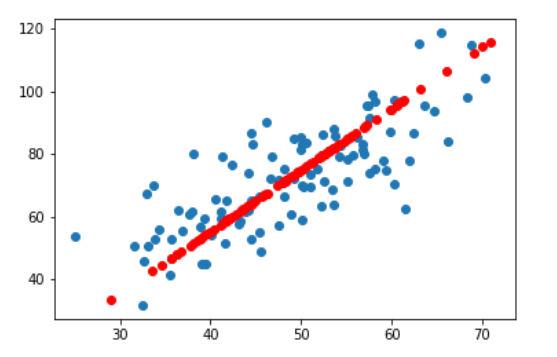## 10. 聚类分析

跳转 —>聚类分析综述与案例实现

展开全文机器学习
• 机器学习-贝叶斯-西瓜分类 对代码的每一行进行了注解，适合初学者使用，主要代码来源是百度ai社区，只是对其进行了注解机器学习
• 本书首先通过实用的案例介绍机器学习的基础知识，然后介绍一些稍微复杂的机器学习算法，例如支持向量机、极端随机森林、隐马尔可夫模型、条件随机场、深度神经网络，等等。本书是为想用机器学习算法开发应用程序的...
• matlab画图代码实例 MatlabML Machine Learning Algorithm implement with Matlab algo: 放machine learning的算法 ...放机器学习模型 util: 一些公共代码 test: 测试代码 lib: 放一些开源的库，例如libsvm
• 主要介绍了Python机器学习k-近邻算法(K Nearest Neighbor),结合实例形式分析了k-近邻算法的原理、操作步骤、相关实现与使用技巧,需要的朋友可以参考下
• 简介k近邻（KNN)是一种基本分类与回归方法。其思路如下：给一个训练数据集和一个新的实例，在训练数据集中找出与这个新实例最近的 个训练实例，然后统计最近的 个训机器学习 算法
• 课程紧密结合实例机器学习领域绝大多数常用算法（涉及监督学习、无监督学习、增强学习、特征工程、深度学习等几乎全领域的数据智能算法）的原理、各算法使用、真实工程中使用进行讲解；除每一种算法都结合程序实例...机器学习 人工智能
• 机器学习是一门多领域交叉学科，涉及概率论、统计学、逼近论、凸分析、算法复杂度理论等多门学科。专门研究计算机怎样模拟或实现人类的学习行为，以获取新的知识或技能，重新组织已有的知识结构使之不断改善自身的...

目录

机器学习

机器学习的步骤

01  线性回归

02 逻辑回归

03 线性判别分析

04 分类和回归树

05 朴素贝叶斯

06 K近邻

07 学习矢量量化

08 支持向量机

09 bagging和随机森林

每文一语

### 机器学习

机器学习是一门多领域交叉学科，涉及概率论、统计学、逼近论凸分析算法复杂度理论等多门学科。专门研究计算机怎样模拟或实现人类的学习行为，以获取新的知识或技能，重新组织已有的知识结构使之不断改善自身的性能。它是人工智能的基础，更是我们现在社会进步的源泉。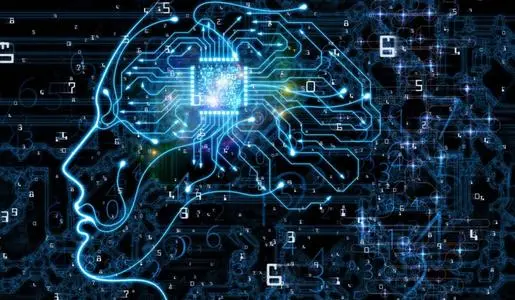随着社会的进步和发展，我们在科技领域不断的创新，当人类的第一台计算机诞生之后，技术的迭代就已经被装上了风火轮了。我们希望计算机可以帮助我们计算我们人类无法涉及到的领域，但是野心永远是人类进化的动力，我们还希望我们的机器可以帮助我们解决我们的工作，那么机器学习就应运而生了。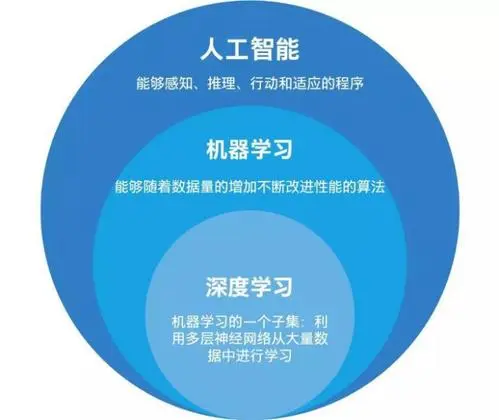人工智能是追求目标，机器学习是实现手段，深度学习是其中一种方法

### 机器学习的步骤

作为一个初学者，机器学习需要掌握怎样的技术呢？首先必须要有一门熟练的编程语言

Python，Java，R语言.....其实Python主要在一些大数据集模型背后占有强大的优势，而R语言在科研统计比较的吃香，因为R语言拥有很多个第三方程序包，利用少量简洁的代码就可以解决我们的科研工作。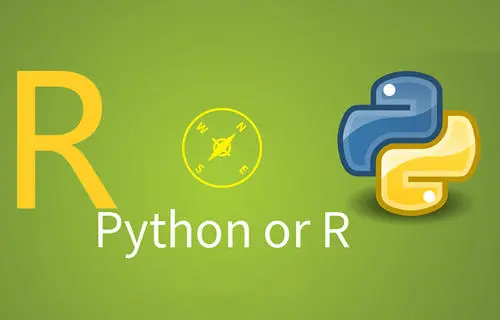1. 选择一个合适的模型，这通常需要依据实际问题而定，针对不同的问题和任务需要选取恰当的模型，模型就是一组函数的集合。
2、判断一个函数的好坏，这需要确定一个衡量标准，也就是我们通常说的损失函数（Loss Function），损失函数的确定也需要依据具体问题而定，如回归问题一般采用欧式距离，分类问题一般采用交叉熵代价函数。
3、找出“最好”的函数，如何从众多函数中最快的找出“最好”的那一个，这一步是最大的难点，做到又快又准往往不是一件容易的事情。常用的方法有梯度下降算法，最小二乘法等和其他一些技巧（tricks）。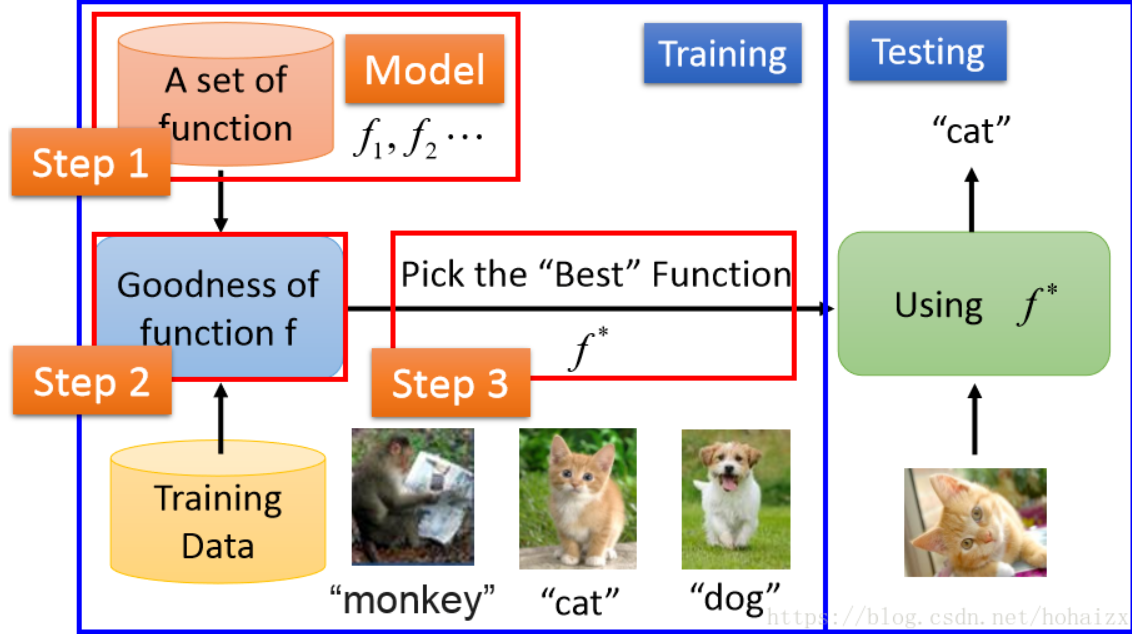这就是为什么我们说机器学习，底层基础是数学理论，其他的应该在编程上面下功夫

差不多认识了我们机器学习，接下来我们就开始从机器学习最常见的10大算法入门，介绍它们的概念知识，最后我们将开启机器学习的大门，详细记录我们学习过程中的点点滴滴！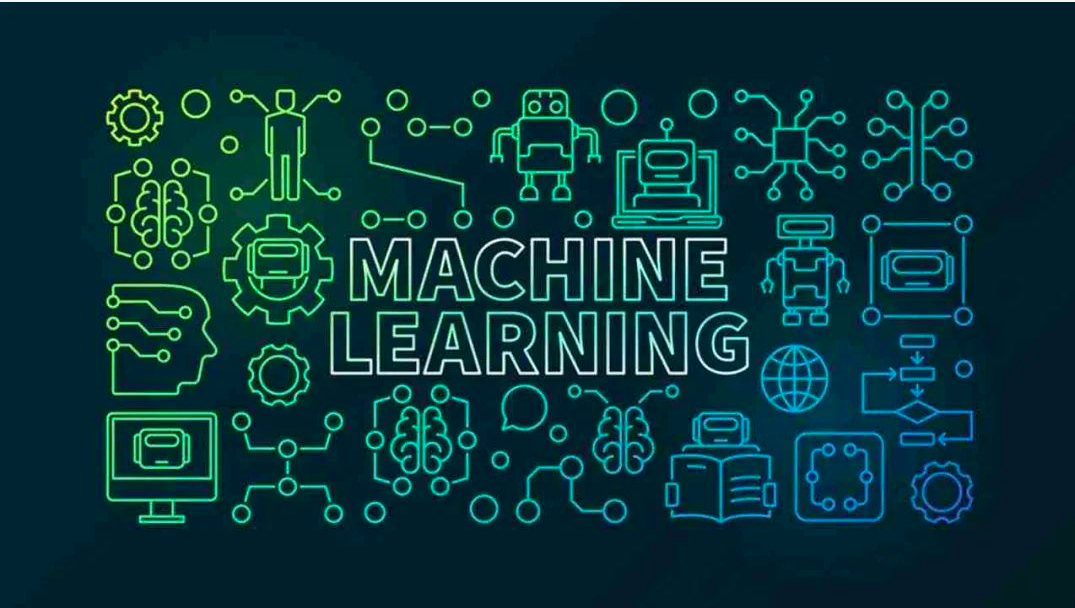在机器学习领域，有种说法叫做“世上没有免费的午餐”，简而言之，它是指没有任何一种算法能在每个问题上都能有最好的效果，这个理论在监督学习方面体现得尤为重要。

举个例子来说，你不能说神经网络永远比决策树好，反之亦然。模型运行被许多因素左右，例如数据集的大小和结构。

因此，你应该根据你的问题尝试许多不同的算法，同时使用数据测试集来评估性能并选出最优项。

当然，你尝试的算法必须和你的问题相切合，其中的门道便是机器学习的主要任务。打个比方，如果你想打扫房子，你可能会用到吸尘器、扫帚或者拖把，但你肯定不会拿把铲子开始挖坑吧。这个道理很多人都懂，哈哈哈。

### 01  线性回归

线性回归可能是统计学和机器学习中最知名和最易理解的算法之一。

由于预测建模主要关注最小化模型的误差，或者以可解释性为代价来做出最准确的预测。 我们会从许多不同领域借用、重用和盗用算法，其中涉及一些统计学知识。

线性回归用一个等式表示，通过找到输入变量的特定权重（B），来描述输入变量（x）与输出变量（y）之间的线性关系。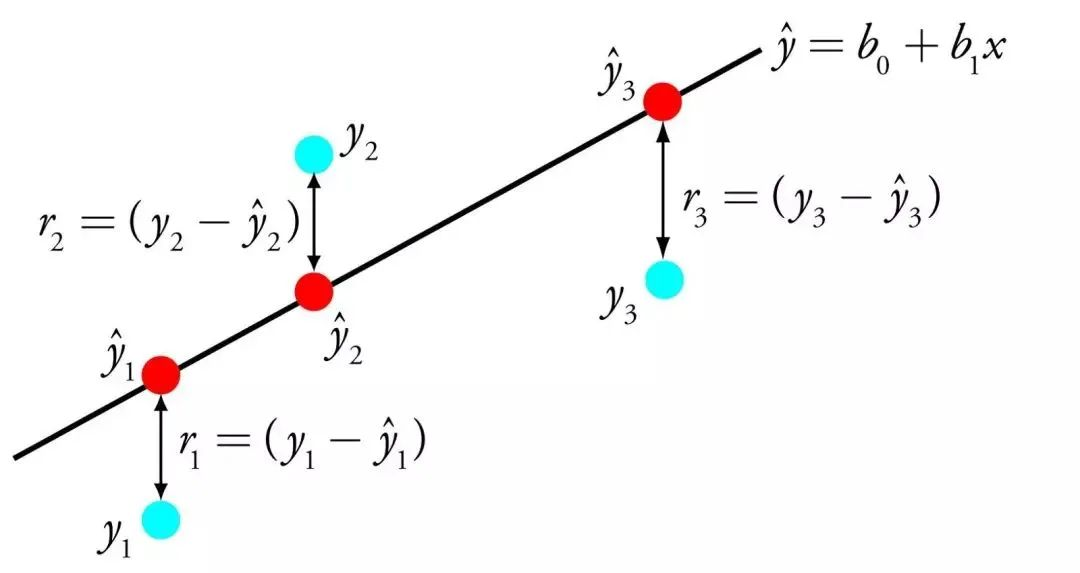Linear Regression

举例：y = B0 + B1 * x

给定输入x，我们将预测y，线性回归学习算法的目标是找到系数B0和B1的值。

可以使用不同的技术从数据中学习线性回归模型，例如用于普通最小二乘和梯度下降优化的线性代数解。

线性回归已经存在了200多年，并且已经进行了广泛的研究。 如果可能的话，使用这种技术时的一些经验法则是去除非常相似（相关）的变量并从数据中移除噪声。 这是一种快速简单的技术和良好的第一种算法。

### 02 逻辑回归

逻辑回归是机器学习从统计领域借鉴的另一种技术。 这是二分类问题的专用方法（两个类值的问题）。

逻辑回归与线性回归类似，这是因为两者的目标都是找出每个输入变量的权重值。 与线性回归不同的是，输出的预测值得使用称为逻辑函数的非线性函数进行变换。

逻辑函数看起来像一个大S，并能将任何值转换为0到1的范围内。这很有用，因为我们可以将相应规则应用于逻辑函数的输出上，把值分类为0和1（例如，如果IF小于0.5，那么 输出1）并预测类别值。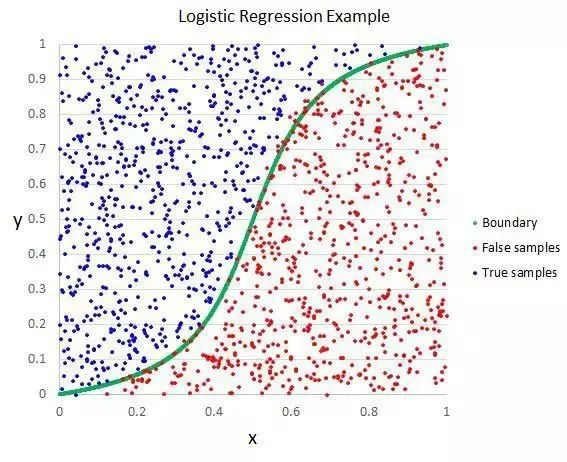Logistic Regression

由于模型的特有学习方式，通过逻辑回归所做的预测也可以用于计算属于类0或类1的概率。这对于需要给出许多基本原理的问题十分有用。

与线性回归一样，当你移除与输出变量无关的属性以及彼此非常相似（相关）的属性时，逻辑回归确实会更好。 这是一个快速学习和有效处理二元分类问题的模型。

### 03 线性判别分析

传统的逻辑回归仅限于二分类问题。 如果你有两个以上的类，那么线性判别分析算法（Linear Discriminant Analysis，简称LDA）是首选的线性分类技术。

LDA的表示非常简单。 它由你的数据的统计属性组成，根据每个类别进行计算。 对于单个输入变量，这包括：

• 每类的平均值。

• 跨所有类别计算的方差。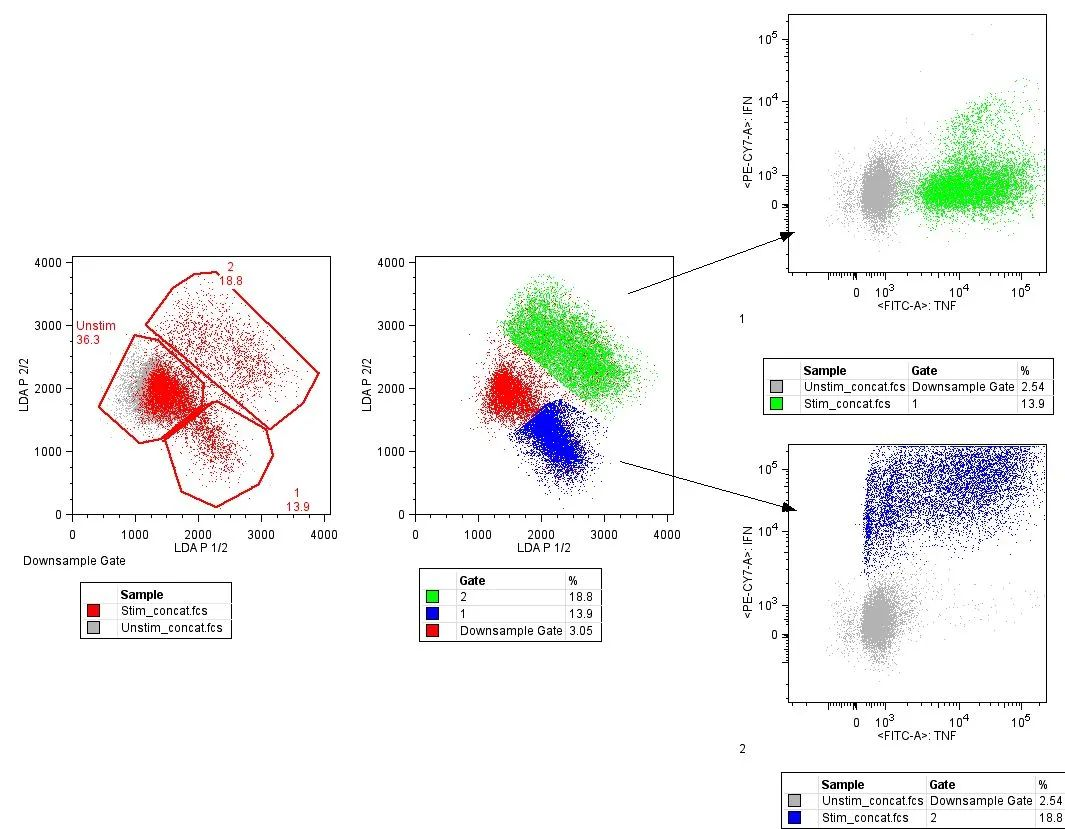Linear Discriminant Analysis

LDA通过计算每个类的判别值并对具有最大值的类进行预测来进行。该技术假定数据具有高斯分布（钟形曲线），因此最好先手动从数据中移除异常值。这是分类预测建模问题中的一种简单而强大的方法。

### 04 分类和回归树

决策树是机器学习的一种重要算法。

决策树模型可用二叉树表示。对，就是来自算法和数据结构的二叉树，没什么特别。 每个节点代表单个输入变量（x）和该变量上的左右孩子（假定变量是数字）。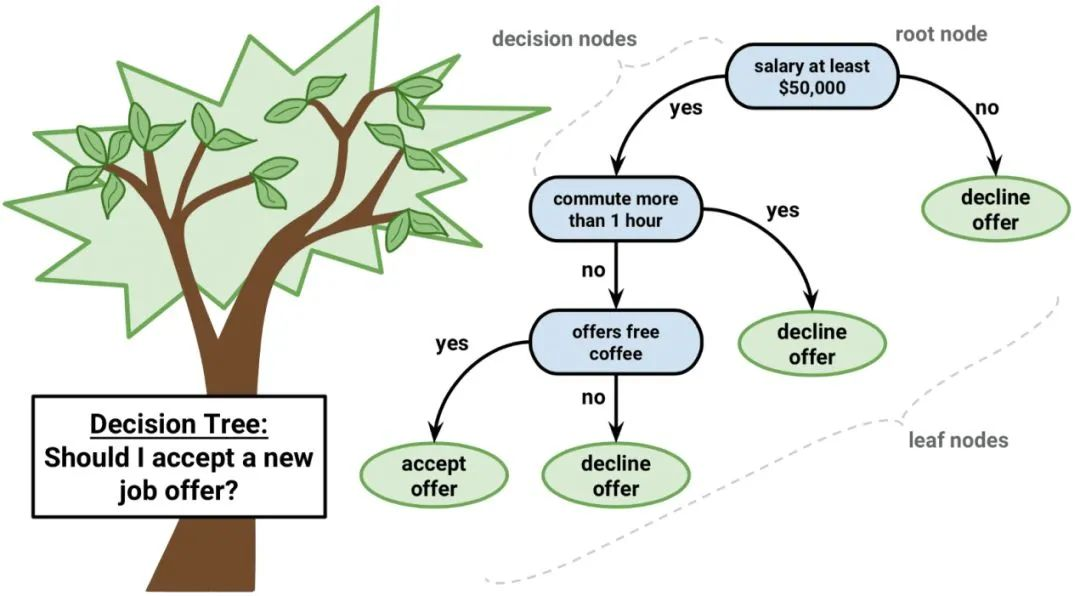Decision Tree

树的叶节点包含用于进行预测的输出变量（y）。 预测是通过遍历树进行的，当达到某一叶节点时停止，并输出该叶节点的类值。

决策树学习速度快，预测速度快。 对于许多问题也经常预测准确，并且你不需要为数据做任何特殊准备。

### 05 朴素贝叶斯

朴素贝叶斯是一种简单但极为强大的预测建模算法。

该模型由两种类型的概率组成，可以直接从你的训练数据中计算出来：1）每个类别的概率; 2）给定的每个x值的类别的条件概率。 一旦计算出来，概率模型就可以用于使用贝叶斯定理对新数据进行预测。 当你的数据是数值时，通常假设高斯分布（钟形曲线），以便可以轻松估计这些概率。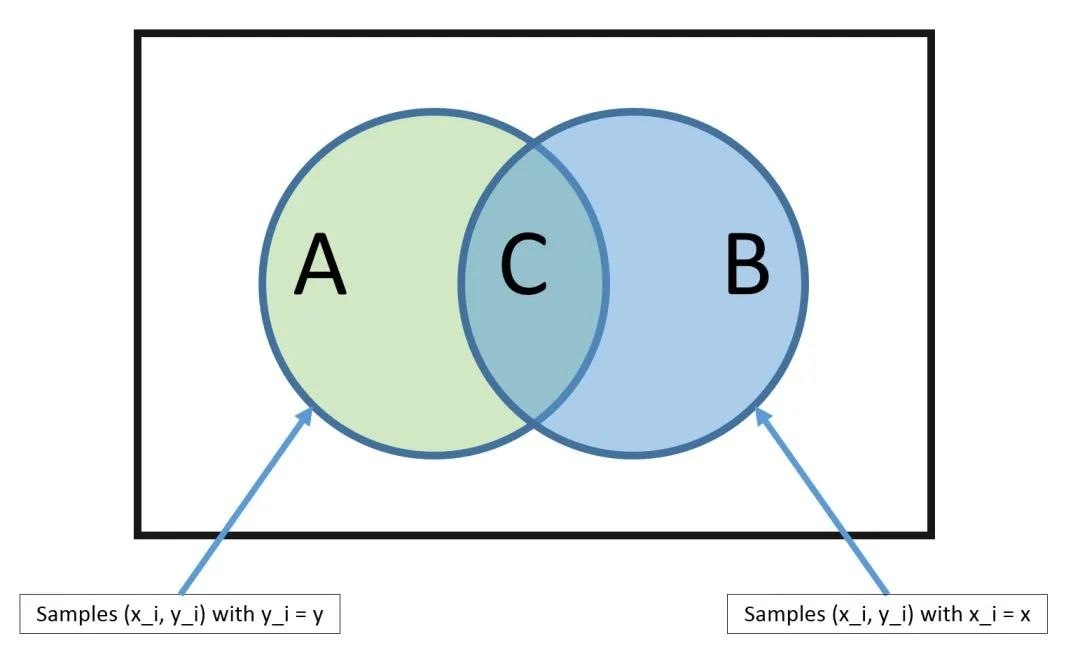Bayes Theorem

朴素贝叶斯被称为朴素的原因，在于它假设每个输入变量是独立的。 这是一个强硬的假设，对于真实数据来说是不切实际的，但该技术对于大范围内的复杂问题仍非常有效。

### 06 K近邻

KNN算法非常简单而且非常有效。KNN的模型用整个训练数据集表示。 是不是特简单？

通过搜索整个训练集内K个最相似的实例（邻居），并对这些K个实例的输出变量进行汇总，来预测新的数据点。 对于回归问题，新的点可能是平均输出变量，对于分类问题，新的点可能是众数类别值。

成功的诀窍在于如何确定数据实例之间的相似性。如果你的属性都是相同的比例，最简单的方法就是使用欧几里德距离，它可以根据每个输入变量之间的差直接计算。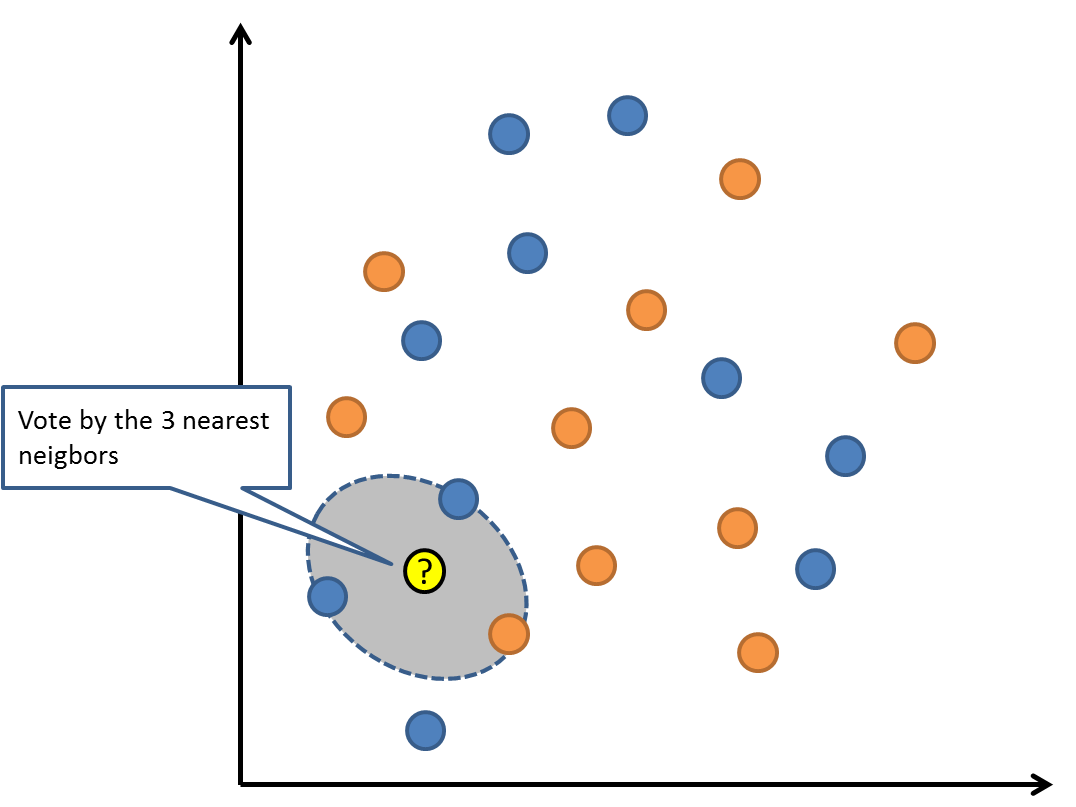K-Nearest Neighbors

KNN可能需要大量的内存或空间来存储所有的数据，但只有在需要预测时才会执行计算（或学习）。 你还可以随时更新和管理你的训练集，以保持预测的准确性。

距离或紧密度的概念可能会在高维环境（大量输入变量）下崩溃，这会对算法造成负面影响。这类事件被称为维度诅咒。它也暗示了你应该只使用那些与预测输出变量最相关的输入变量。

### 07 学习矢量量化

K-近邻的缺点是你需要维持整个训练数据集。 学习矢量量化算法（或简称LVQ）是一种人工神经网络算法，允许你挂起任意个训练实例并准确学习他们。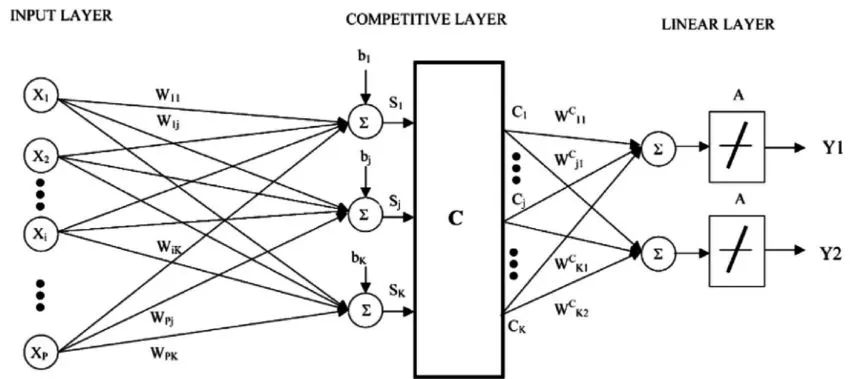Learning Vector Quantization

LVQ用codebook向量的集合表示。开始时随机选择向量，然后多次迭代，适应训练数据集。 在学习之后，codebook向量可以像K-近邻那样用来预测。 通过计算每个codebook向量与新数据实例之间的距离来找到最相似的邻居（最佳匹配），然后返回最佳匹配单元的类别值或在回归情况下的实际值作为预测。 如果你把数据限制在相同范围（如0到1之间），则可以获得最佳结果。

如果你发现KNN在您的数据集上给出了很好的结果，请尝试使用LVQ来减少存储整个训练数据集的内存要求。

### 08 支持向量机

支持向量机也许是最受欢迎和讨论的机器学习算法之一。

超平面是分割输入变量空间的线。 在SVM中，会选出一个超平面以将输入变量空间中的点按其类别（0类或1类）进行分离。在二维空间中可以将其视为一条线，所有的输入点都可以被这条线完全分开。SVM学习算法就是要找到能让超平面对类别有最佳分离的系数。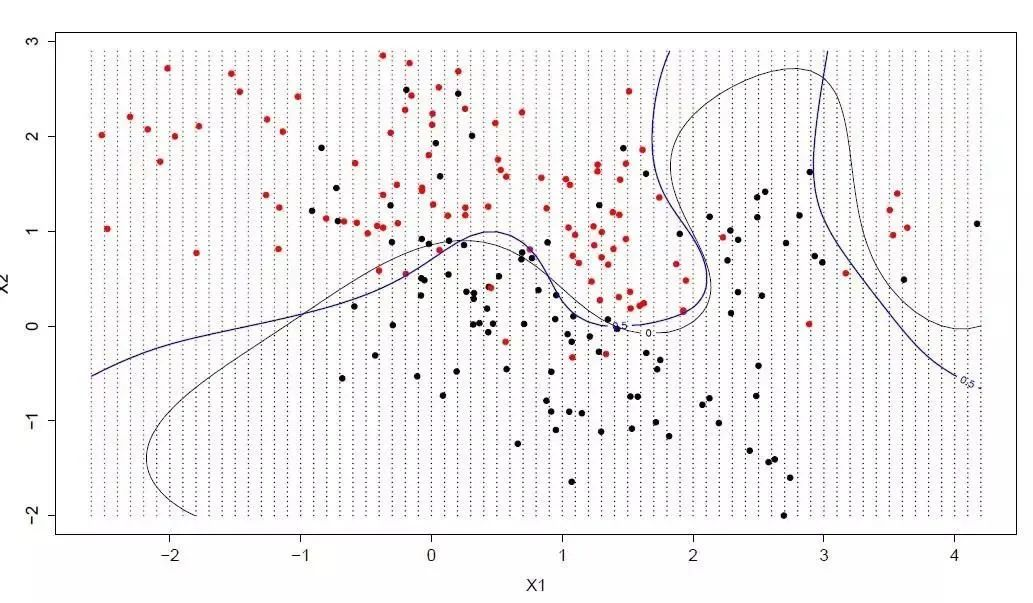Support Vector Machine

超平面和最近的数据点之间的距离被称为边界，有最大边界的超平面是最佳之选。同时，只有这些离得近的数据点才和超平面的定义和分类器的构造有关，这些点被称为支持向量，他们支持或定义超平面。在具体实践中，我们会用到优化算法来找到能最大化边界的系数值。

SVM可能是最强大的即用分类器之一，在你的数据集上值得一试。

### 09 bagging和随机森林

随机森林是最流行和最强大的机器学习算法之一。 它是一种被称为Bootstrap Aggregation或Bagging的集成机器学习算法。

bootstrap是一种强大的统计方法，用于从数据样本中估计某一数量，例如平均值。 它会抽取大量样本数据，计算平均值，然后平均所有平均值，以便更准确地估算真实平均值。

在bagging中用到了相同的方法，但最常用到的是决策树，而不是估计整个统计模型。它会训练数据进行多重抽样，然后为每个数据样本构建模型。当你需要对新数据进行预测时，每个模型都会进行预测，并对预测结果进行平均，以更好地估计真实的输出值。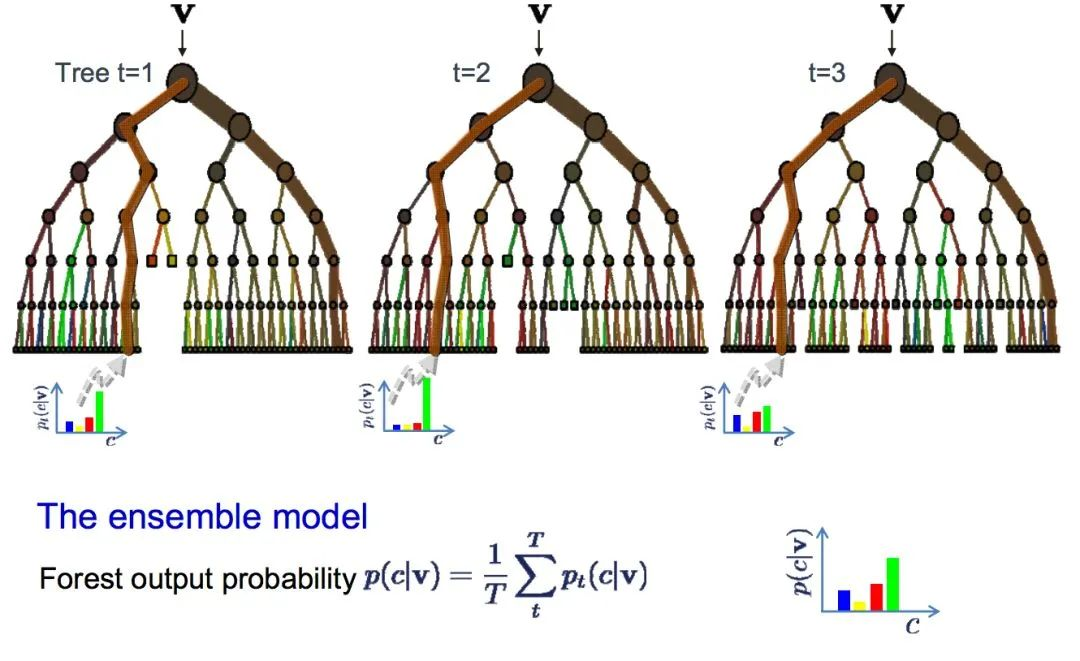Random Forest

随机森林是对决策树的一种调整，相对于选择最佳分割点，随机森林通过引入随机性来实现次优分割。

因此，为每个数据样本创建的模型之间的差异性会更大，但就自身意义来说依然准确无误。结合预测结果可以更好地估计正确的潜在输出值。

如果你使用高方差算法（如决策树）获得良好结果，那么加上这个算法后效果会更好。

Boosting是一种从一些弱分类器中创建一个强分类器的集成技术。 它先由训练数据构建一个模型，然后创建第二个模型来尝试纠正第一个模型的错误。 不断添加模型，直到训练集完美预测或已经添加到数量上限。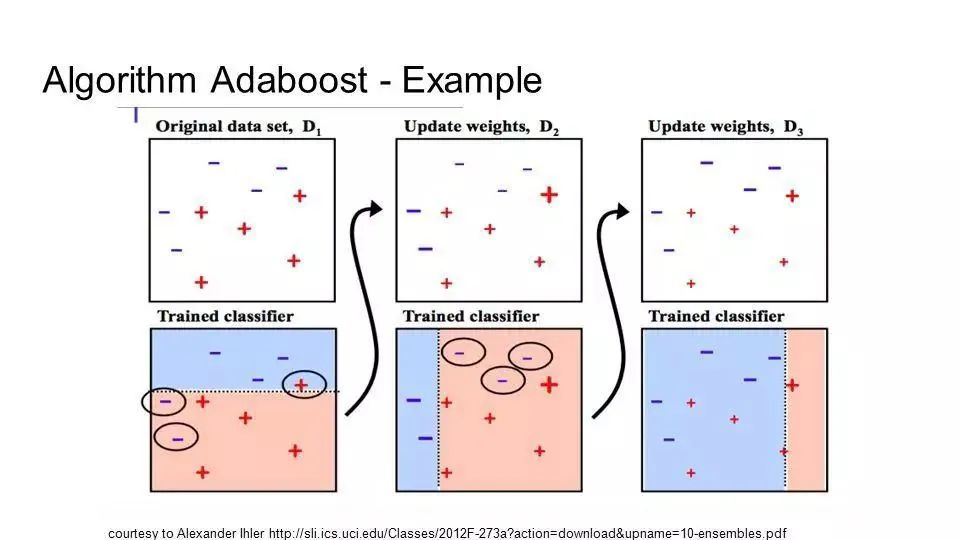• 数据的大小，质量和性质;

• 可用的计算时间;

• 任务的紧迫性;

• 你想要对数据做什么。

是我们选择那种机器学习算法的关键

scikit-learn作为一个丰富的python机器学习库，实现了绝大多数机器学习的算法，有相当多的人在使用，于是我这里很无耻地把machine learning cheat sheet for sklearn搬过来了，原文可以看这里。哈哈，既然讲机器学习，我们就用机器学习的语言来解释一下，这是针对实际应用场景的各种条件限制，对scikit-learn里完成的算法构建的一颗决策树，每一组条件都是对应一条路径，能找到相对较为合适的一些解决方法，具体如下：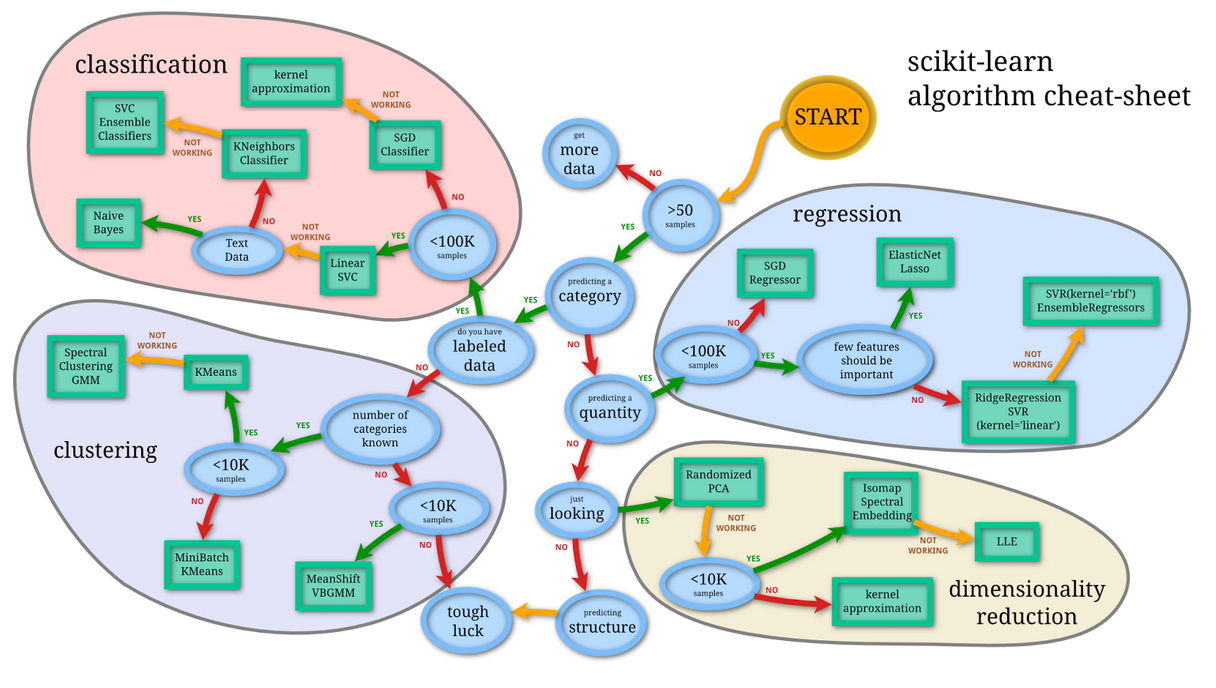### 每文一语

既然选择，就像当时一样，不问未来

展开全文机器学习 人工智能
• 本文介绍了10中常用的机器算法原理，包括有监督学习和无监督学习，通过本文档可以对10中机器学习有基本的认识。
• ## 十大机器学习算法

万次阅读 多人点赞 2022-03-08 22:55:09
初学者应该知道的十大机器学习算法 来源：https://builtin.com/data-science/tour-top-10-algorithms-machine-learning-newbies 机器学习算法被描述为学习一个目标函数 (f)，它最好地将输入变量 (X) 映射到输出...机器学习
•机器学习 近邻算法
• 利用高效的可复用Python代码来阐释如何处理统计数据...通过各种实例，可从中学会机器学习的核心算法，并能将其运用于一些策略性任务中，如分类、预测、推荐。另外，还可用它们来实现一些更高级的功能，如汇总和简化等。机器学习实战源码
• 人工智能-机器学习-关联规则分析-Apriori算法实例-挖掘电影导演的关联规则
• 我相信很多人跟我一样，学习机器学习和数据科学的第一个算法是线性回归，它简单易懂。由于其功能有限，它不太可能成为工作中的最佳选择。大多数情况下，线性回归被用作基线模型来评估和比较研究中的新方法。 在处理...python 人工智能 机器学习
•机器学习 人工智能
• 图解AI数学基础：从入门到精通系列教程 图解大数据技术：从入门到精通系列教程 图解机器学习算法：从入门到精通系列教程 机器学习实战：手把手教你玩转机器学习系列 相关文章推荐 Python机器学习算法应用实践 ...机器学习 人工智能 sklearn
• BP神经网络详解与实例 BP算法 神经网络 机器学习 马尔.ppt
• k近邻算法没有显式的学习过程。 1.模型：k近邻法使用的模型对应于对特征空间的划分。 k近邻法中，当训练集、k值、距离度量(如欧式距离)及分类决策规则确定后，对于任何一个新的输入实例，它所属的类唯一地确定。 ......